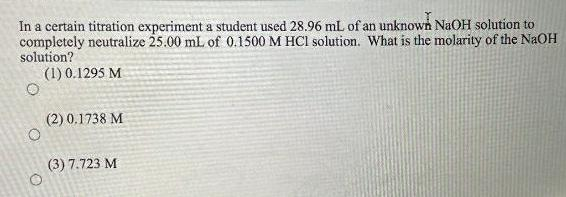Physical Chemistry
Solutions
In a certain titration experiment a student used 28 96 mL of an unknown NaOH solution to completely neutralize 25 00 mL of 0 1500 M HCl solution What is the molarity of the NaOH solution 1 0 1295 M O O 2 0 1738 M 3 7 723 M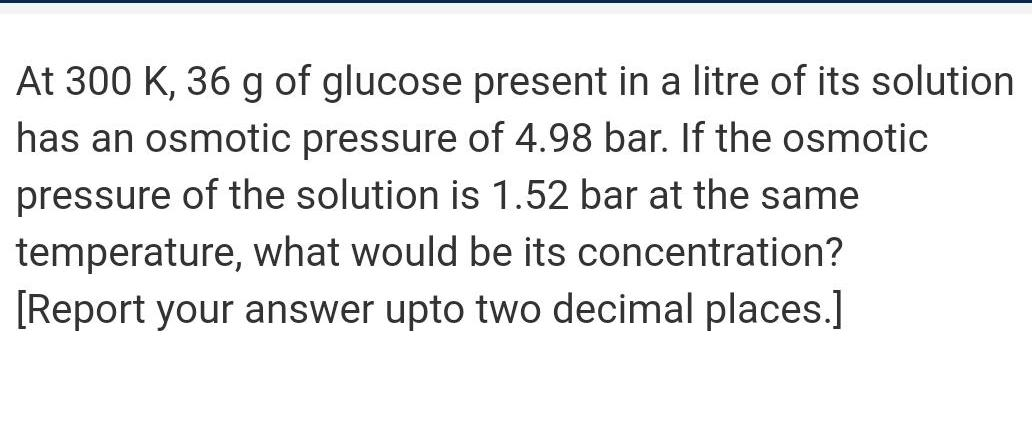Physical Chemistry
Solutions
At 300 K 36 g of glucose present in a litre of its solution has an osmotic pressure of 4 98 bar If the osmotic pressure of the solution is 1 52 bar at the same temperature what would be its concentration Report your answer upto two decimal places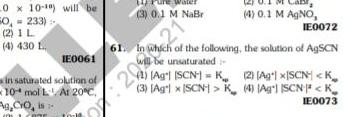Physical Chemistry
Solutions
0 x 10 10 will be 0 233 2 IL 4 430 L IE0061 in saturated solution of 10 mol L At 20 C Ag CrO is 3 0 1 M NaBr 27 IE0072 61 In which of the following the solution of AgSCN 12 3 20 will be unsaturated 20 4 0 1 M AgNO 1 Ag SCN1K 2 Aglx SCN K 3 Agl x SCN1 K 4 Ag SCN K IE0073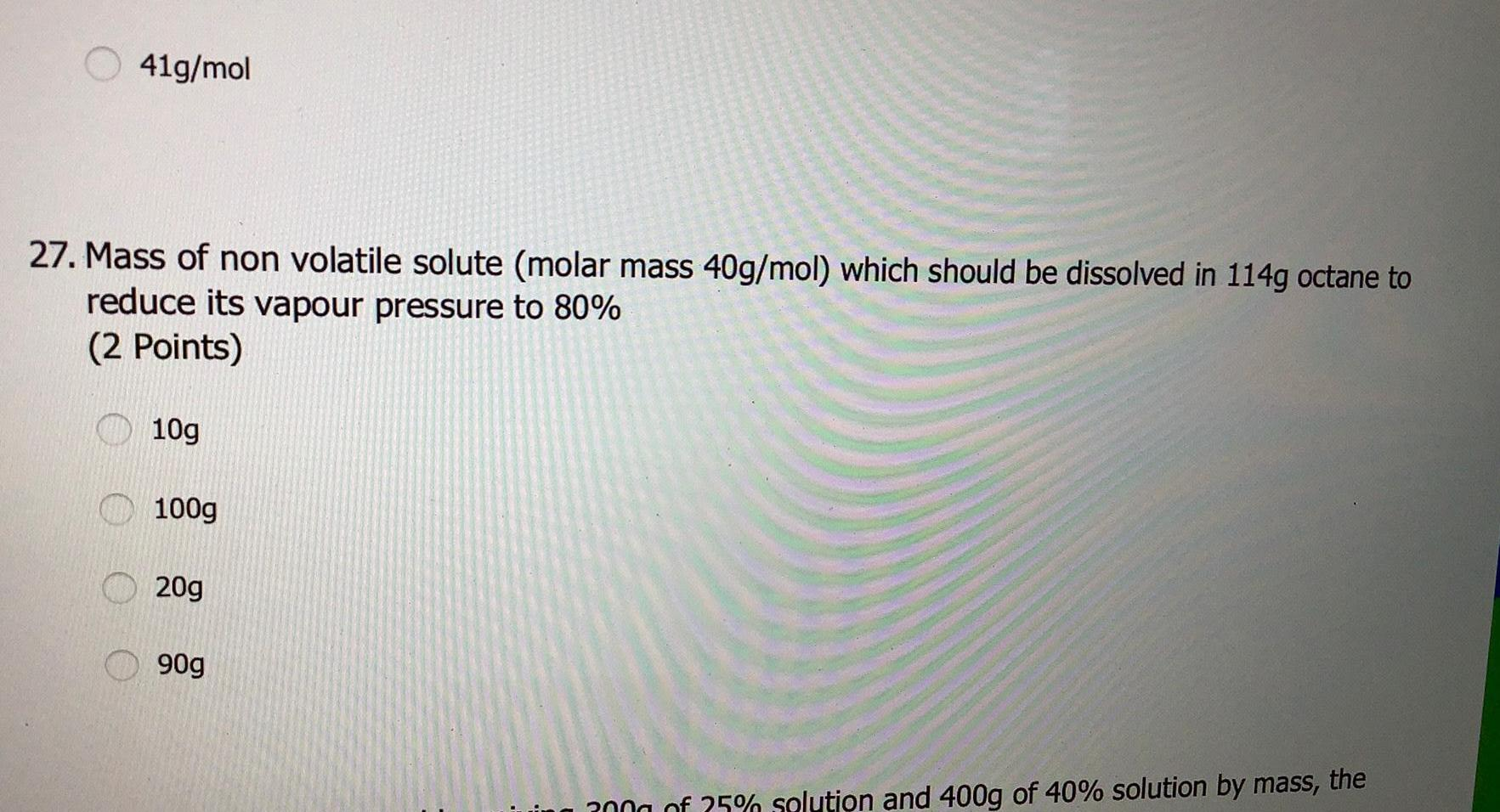Physical Chemistry
Solutions
41g mol 27 Mass of non volatile solute molar mass 40g mol which should be dissolved in 114g octane to reduce its vapour pressure to 80 2 Points 10g 100g 20g 90g 300g of 25 solution and 400g of 40 solution by mass the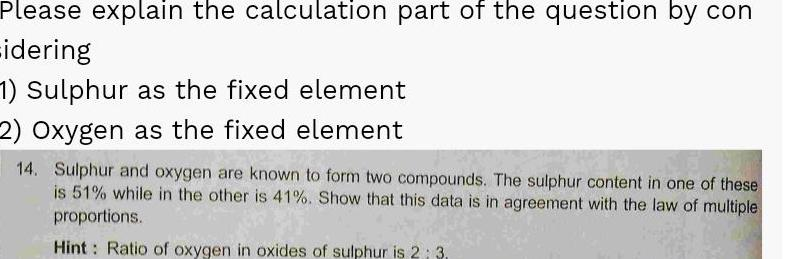Physical Chemistry
Solutions
Please explain the calculation part of the question by con idering 1 Sulphur as the fixed element 2 Oxygen as the fixed element 14 Sulphur and oxygen are known to form two compounds The sulphur content in one of these is 51 while in the other is 41 Show that this data is in agreement with the law of multiple proportions Hint Ratio of oxygen in oxides of sulphur is 2 3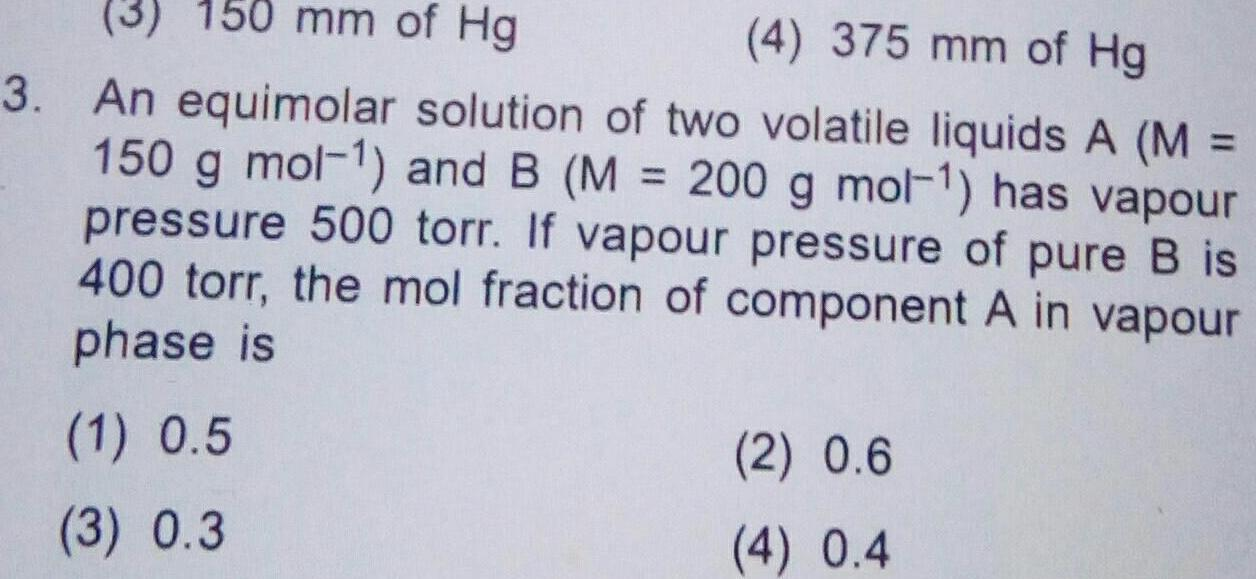Physical Chemistry
Solutions
3 150 mm of Hg 4 375 mm of Hg 3 An equimolar solution of two volatile liquids A M 150 g mol and B M 200 g mol 1 has vapour pressure 500 torr If vapour pressure of pure B is 400 torr the mol fraction of component A in vapour phase is 1 0 5 3 0 3 2 0 6 4 0 4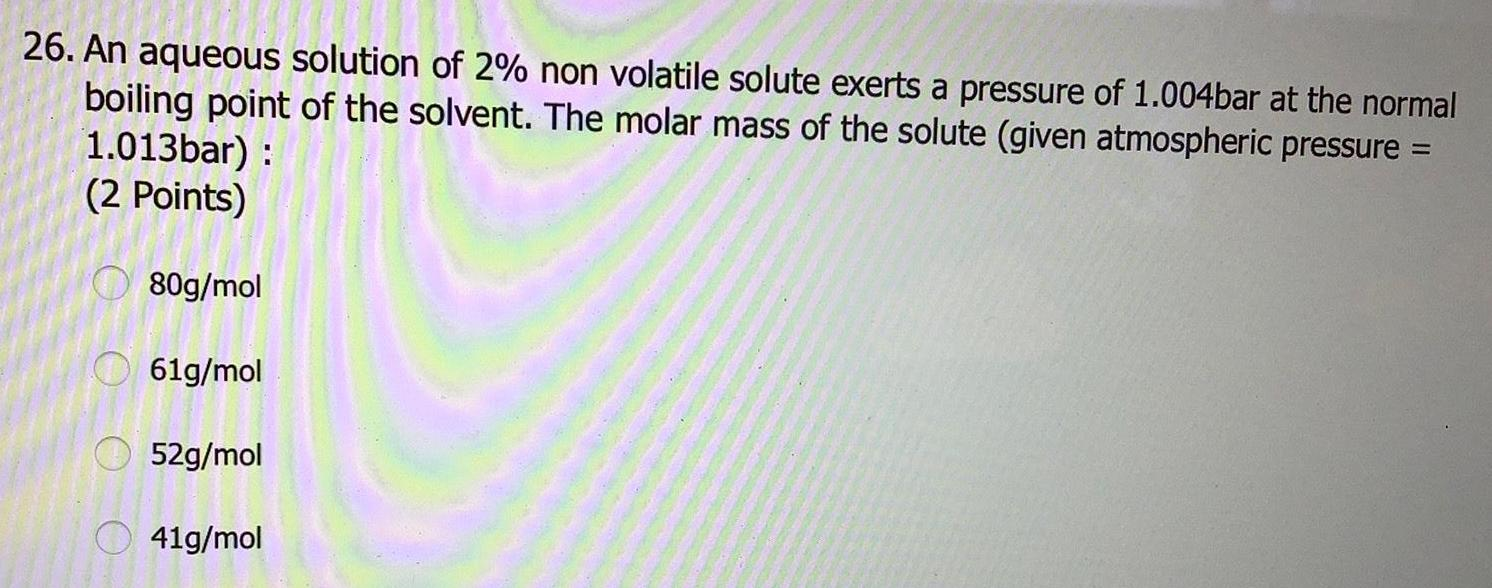Physical Chemistry
Solutions
26 An aqueous solution of 2 non volatile solute exerts a pressure of 1 004bar at the normal boiling point of the solvent The molar mass of the solute given atmospheric pressure 1 013bar 2 Points 80g mol 61g mol 52g mol 41g mol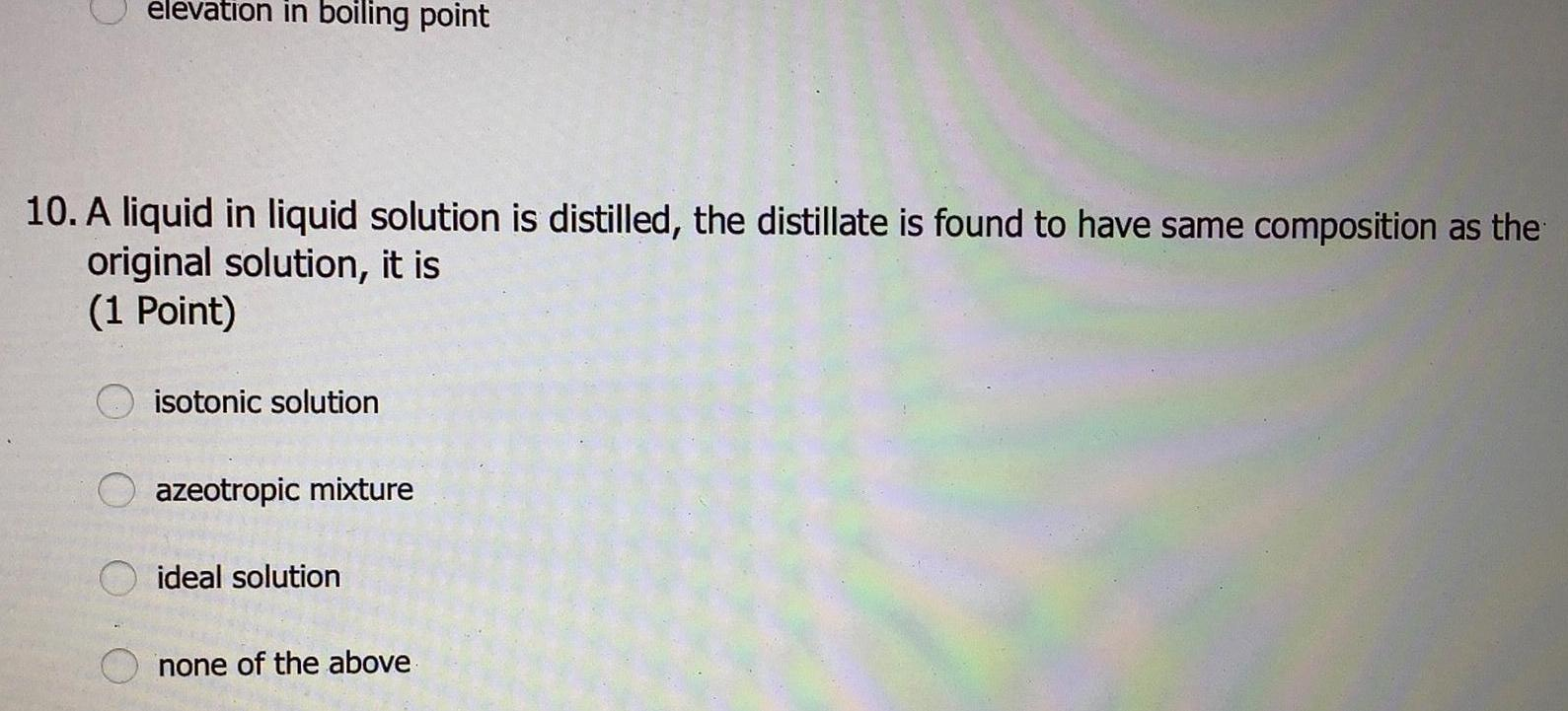Physical Chemistry
Solutions
elevation in boiling point 10 A liquid in liquid solution is distilled the distillate is found to have same composition as the original solution it is 1 Point isotonic solution azeotropic mixture ideal solution none of the above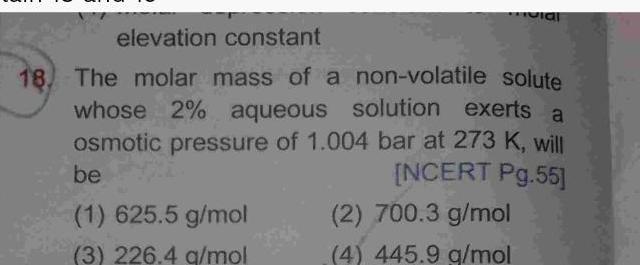Physical Chemistry
Solutions
elevation constant 18 The molar mass of a non volatile solute whose 2 aqueous solution exerts a osmotic pressure of 1 004 bar at 273 K will be NCERT Pg 55 1 625 5 g mol 3 226 4 g mol vial 2 700 3 g mol 4 445 9 g mol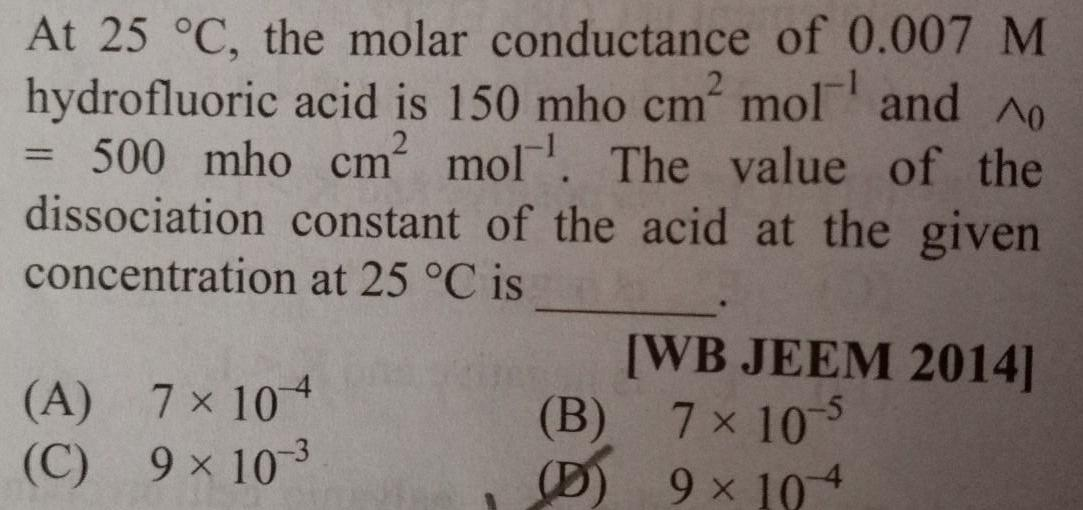Physical Chemistry
Solutions
At 25 C the molar conductance of 0 007 M hydrofluoric acid is 150 mho cm mol and o 500 mho cm mol The value of the dissociation constant of the acid at the given concentration at 25 C is A C 7 104 9 10 3 B D WB JEEM 2014 7 10 5 9 104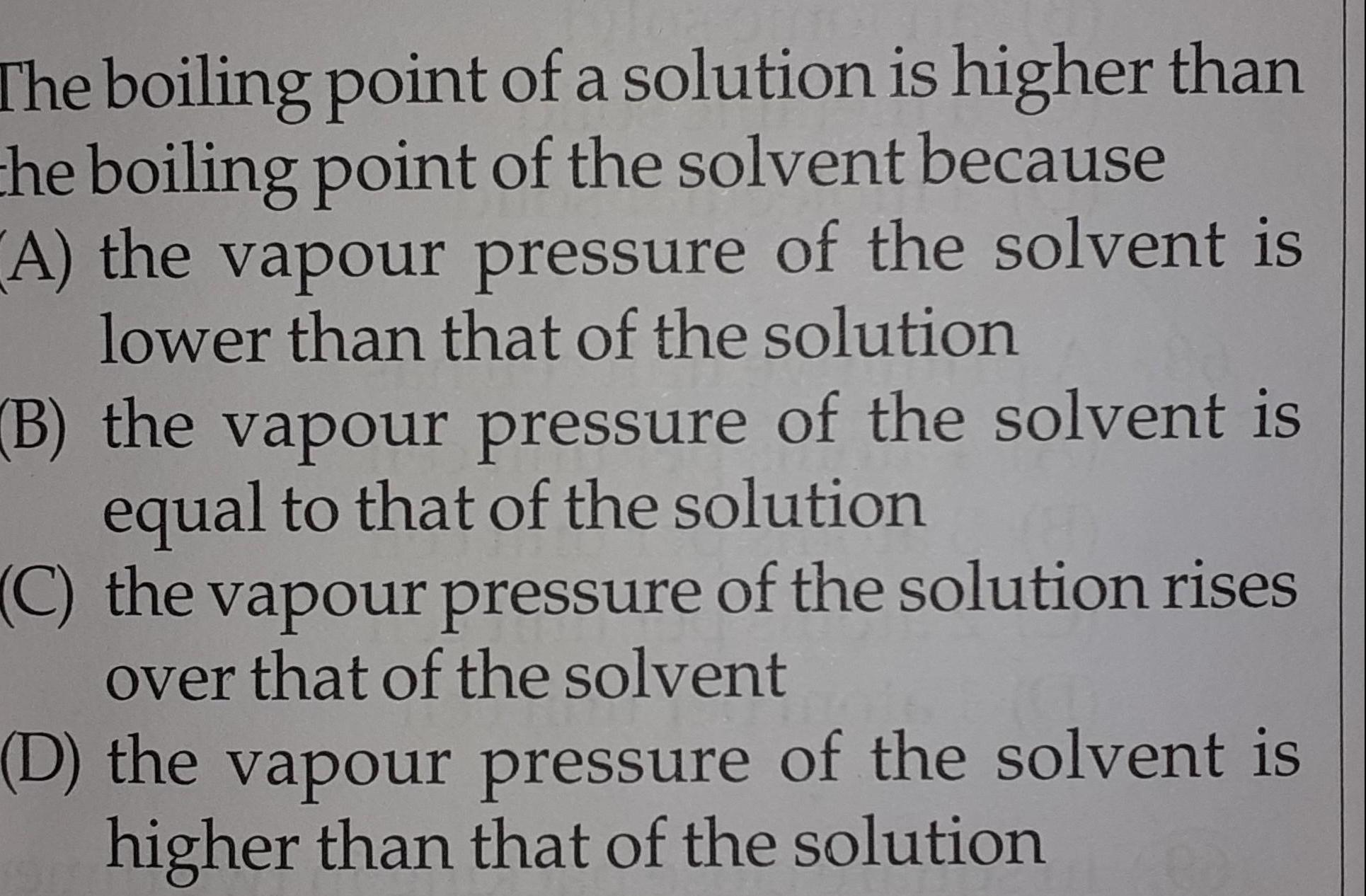Physical Chemistry
Solutions
The boiling point of a solution is higher than the boiling point of the solvent because A the vapour pressure of the solvent is lower than that of the solution B the vapour pressure of the solvent is equal to that of the solution C the vapour pressure of the solution rises over that of the solvent D the vapour pressure of the solvent is higher than that of the solution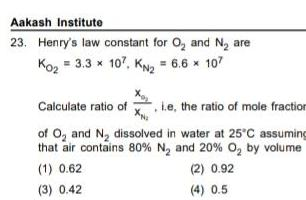Physical Chemistry
Solutions
Aakash Institute 23 Henry s law constant for O and N are Ko 3 3 x 107 KN 6 6 107 Calculate ratio of ie the ratio of mole fraction Xp of O and N dissolved in water at 25 C assuming that air contains 80 N and 20 O by volume 1 0 62 2 0 92 3 0 42 4 0 5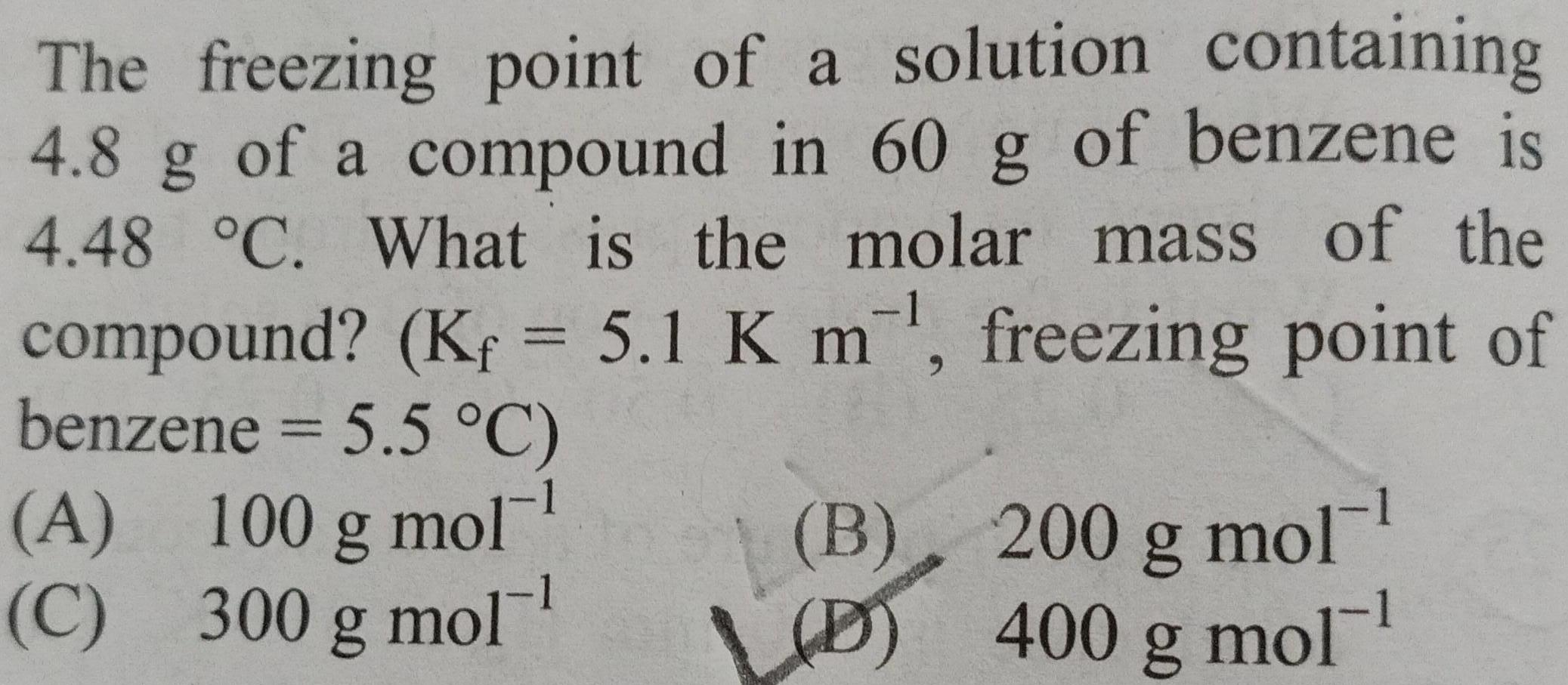Physical Chemistry
Solutions
The freezing point of a solution containing 4 8 g of a compound in 60 g of benzene is 4 48 C What is the molar mass of the compound Kf 5 1 K m freezing point of benzene 5 5 C A 100 g mol 300 g mol C B 200 g mol D 400 g mol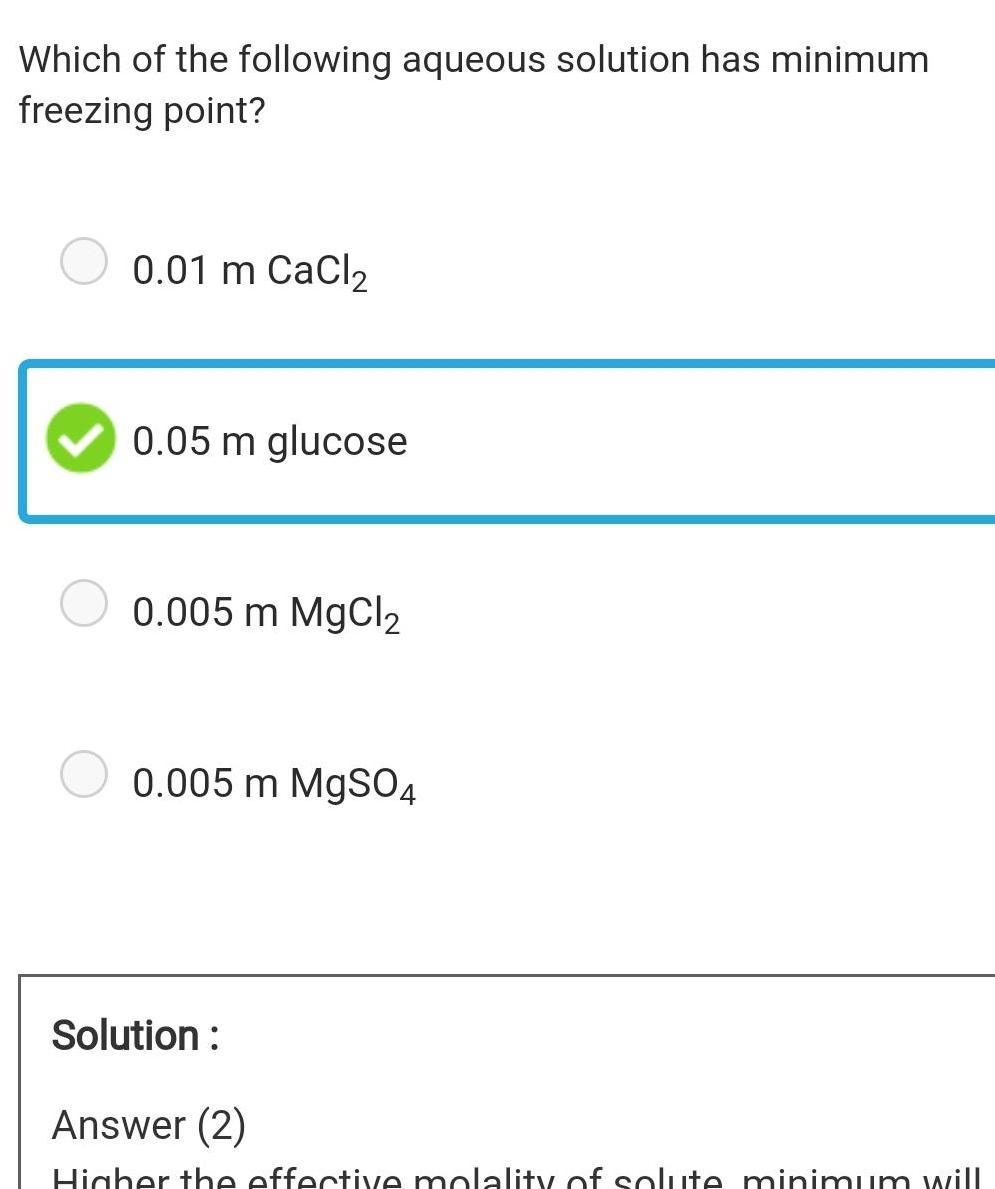Physical Chemistry
Solutions
Which of the following aqueous solution has minimum freezing point 0 01 m CaCl 0 05 m glucose 0 005 m MgCl 0 005 m MgSO4 Solution Answer 2 Higher the effective molality of solute minimum will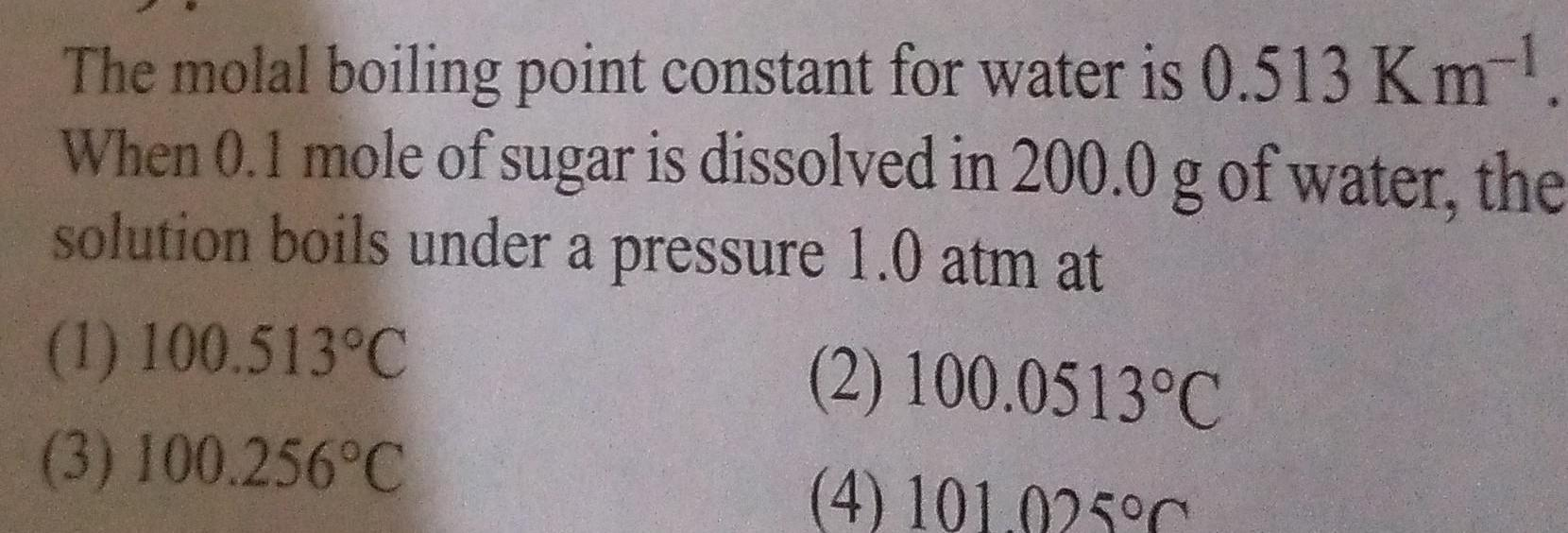Physical Chemistry
Solutions
The molal boiling point constant for water is 0 513 Km When 0 1 mole of sugar is dissolved in 200 0 g of water the solution boils under a pressure 1 0 atm at 2 100 0513 C 4 101 025 C 1 100 513 C 3 100 256 C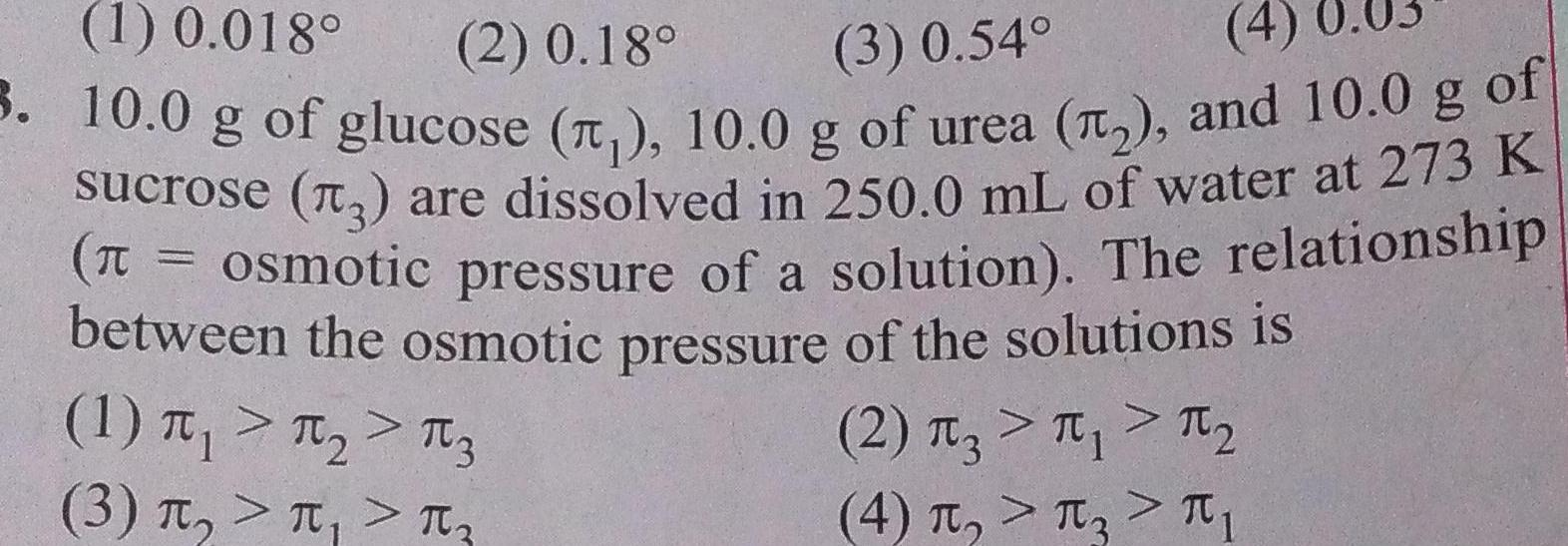Physical Chemistry
Solutions
1 0 018 2 0 18 3 0 54 4 3 10 0 g of glucose 10 0 g of urea and 10 0 g of sucrose are dissolved in 250 0 mL of water at 273 K osmotic pressure of a solution The relationship between the osmotic pressure of the solutions is 2 3 1 TC 1 3 3 4 T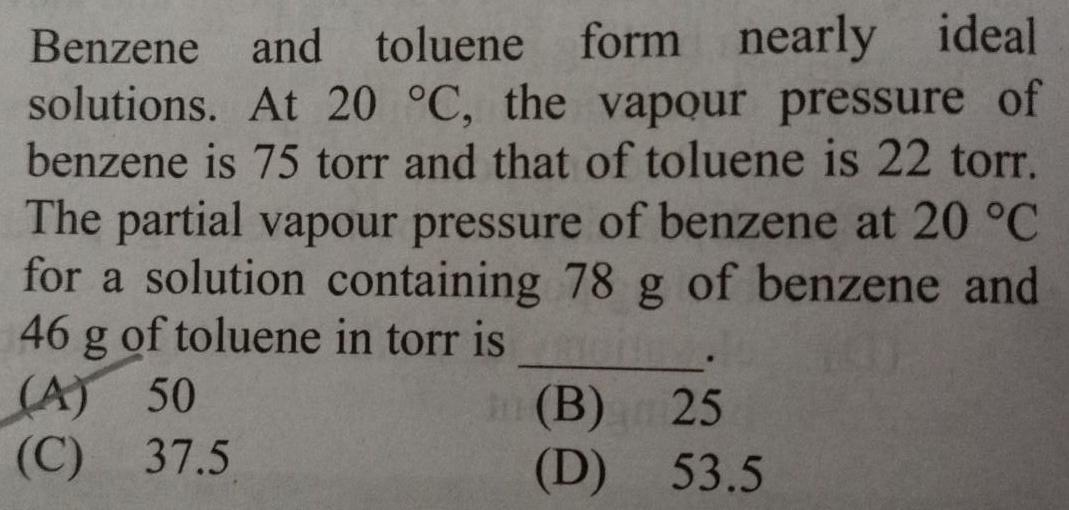Physical Chemistry
Solutions
Benzene and toluene form nearly ideal solutions At 20 C the vapour pressure of benzene is 75 torr and that of toluene is 22 torr The partial vapour pressure of benzene at 20 C for a solution containing 78 g of benzene and 46 g of toluene in torr is A 50 C 37 5 B 25 D 53 5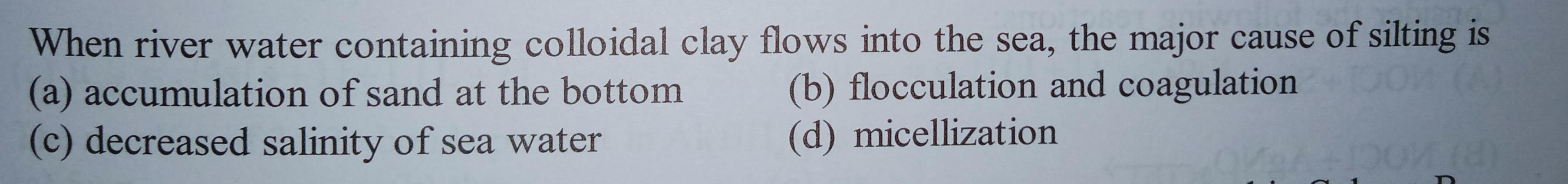Physical Chemistry
Solutions
When river water containing colloidal clay flows into the sea the major cause of silting is a accumulation of sand at the bottom c decreased salinity of sea water b flocculation and coagulation d micellization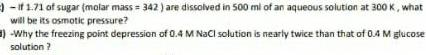Physical Chemistry
Solutions
If 1 71 of sugar molar mass 342 are dissolved in 500 ml of an aqueous solution at 300 K what will be its osmotic pressure 1 Why the freezing point depression of 0 4 M NaCl solution is nearly twice than that of 0 4 M glucose solution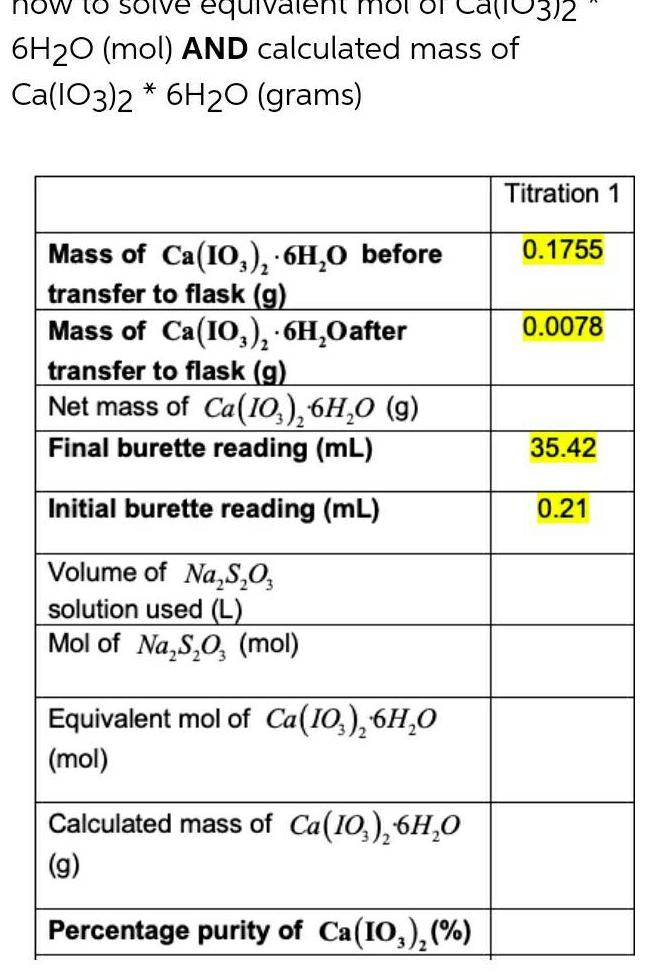Physical Chemistry
Solutions
how to solve equiva 6H20 mol AND calculated mass of Ca 103 2 6H2O grams Mass of Ca 10 6H O before transfer to flask g Mass of Ca 103 6H Oafter transfer to flask g Net mass of Ca 10 6H O 9 Final burette reading mL Initial burette reading mL Volume of Na S O solution used L Mol of Na S O mol Equivalent mol of Ca 10 6H 0 mol Calculated mass of Ca 10 6H 0 g Percentage purity of Ca 10 103 2 Titration 1 0 1755 0 0078 35 42 0 21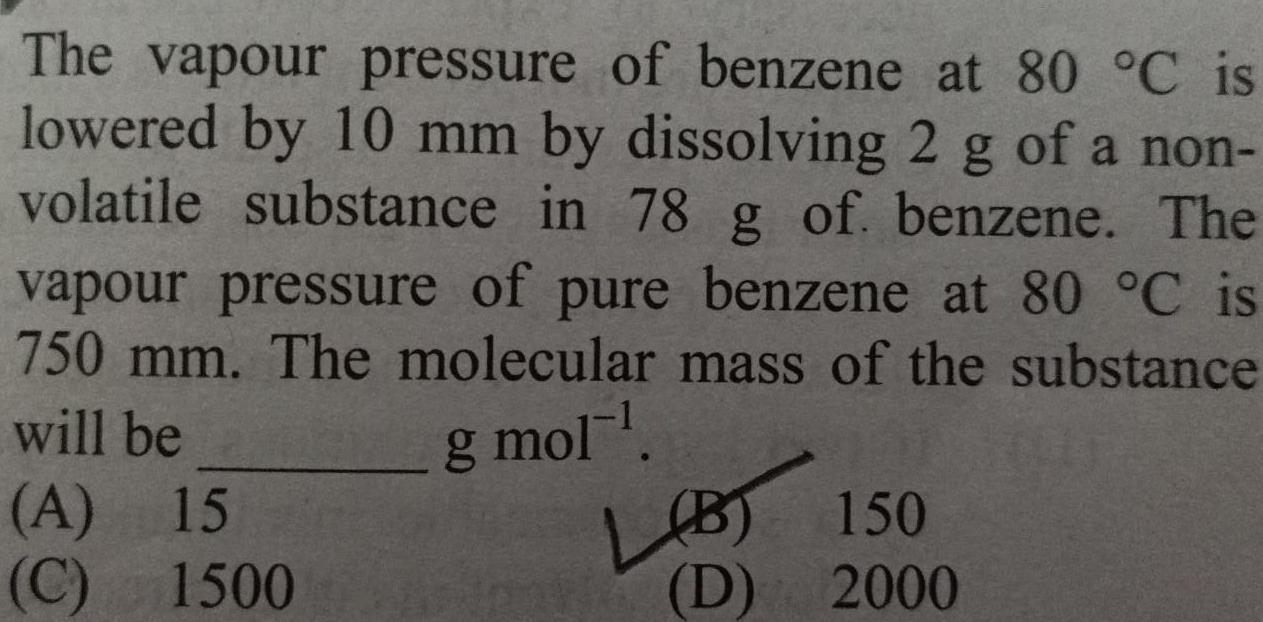Physical Chemistry
Solutions
The vapour pressure of benzene at 80 C is lowered by 10 mm by dissolving 2 g of a non volatile substance in 78 g of benzene The vapour pressure of pure benzene at 80 C is 750 mm The molecular mass of the substance will be g mol A 15 C 1500 LOBT LB 150 D 2000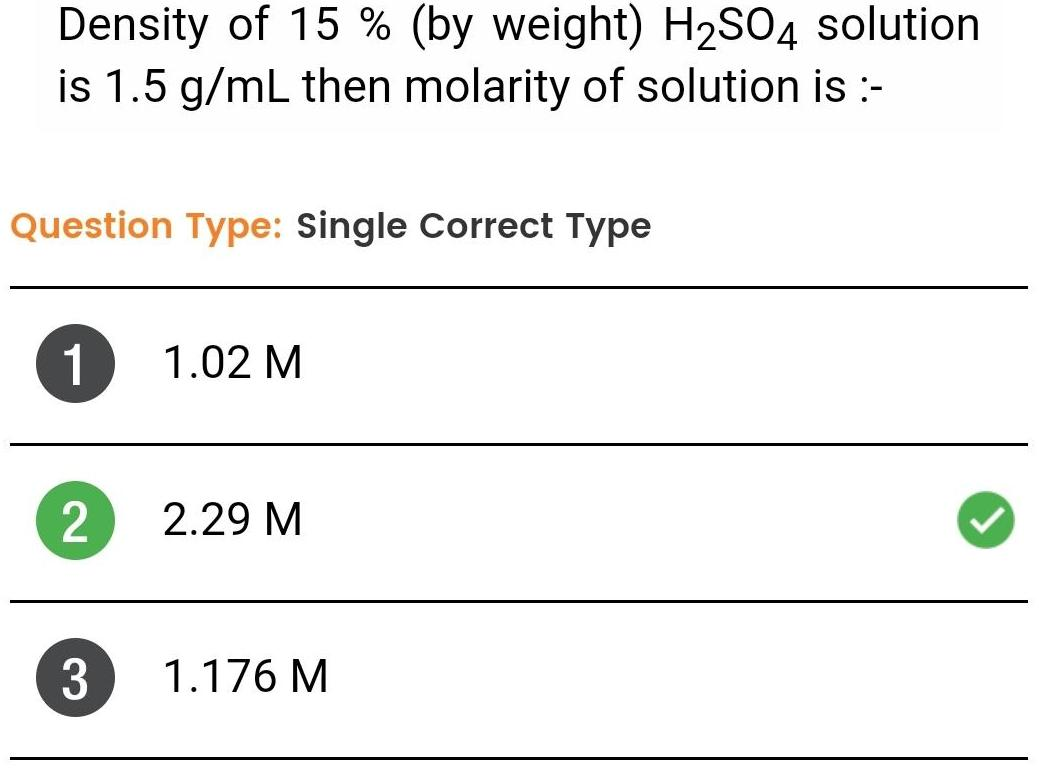Physical Chemistry
Solutions
Density of 15 by weight H SO4 solution is 1 5 g mL then molarity of solution is Question Type Single Correct Type 1 2 3 1 02 M 2 29 M 1 176 M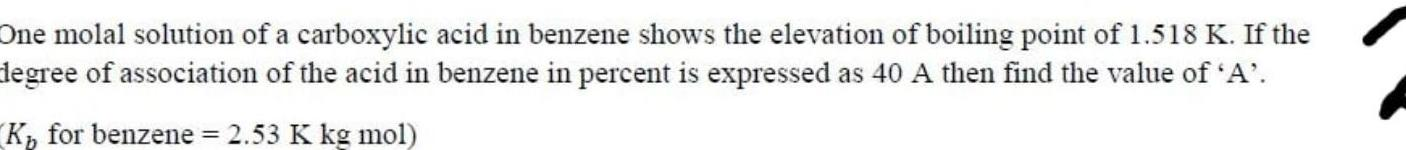Physical Chemistry
Solutions
One molal solution of a carboxylic acid in benzene shows the elevation of boiling point of 1 518 K If the degree of association of the acid in benzene in percent is expressed as 40 A then find the value of A K for benzene 2 53 K kg mol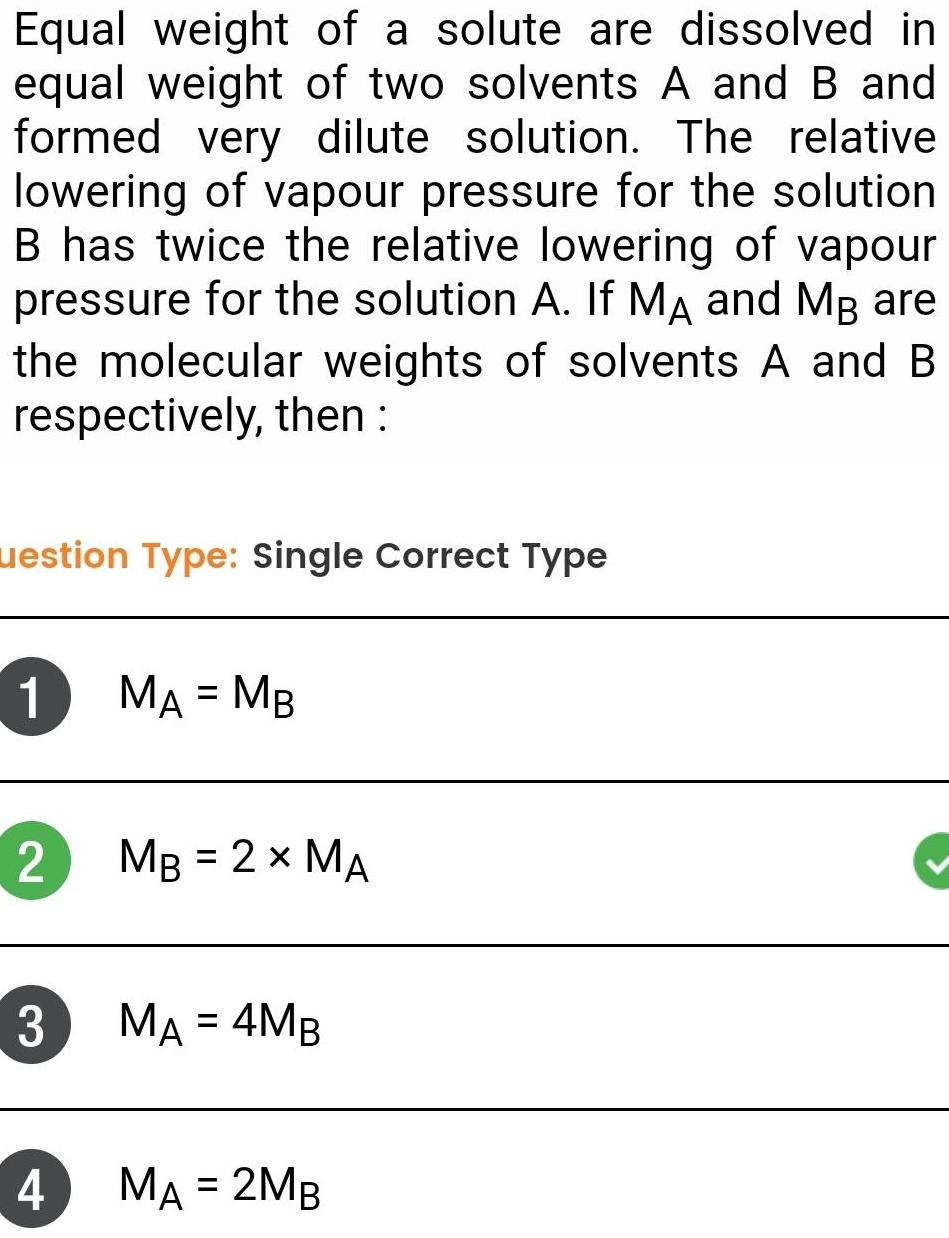Physical Chemistry
Solutions
Equal weight of a solute are dissolved in equal weight of two solvents A and B and formed very dilute solution The relative lowering of vapour pressure for the solution B has twice the relative lowering of vapour pressure for the solution A If MA and MB are the molecular weights of solvents A and B respectively then uestion Type Single Correct Type 1 MA MB 2 MB 2 MA 3 4 MA 4MB MA 2MB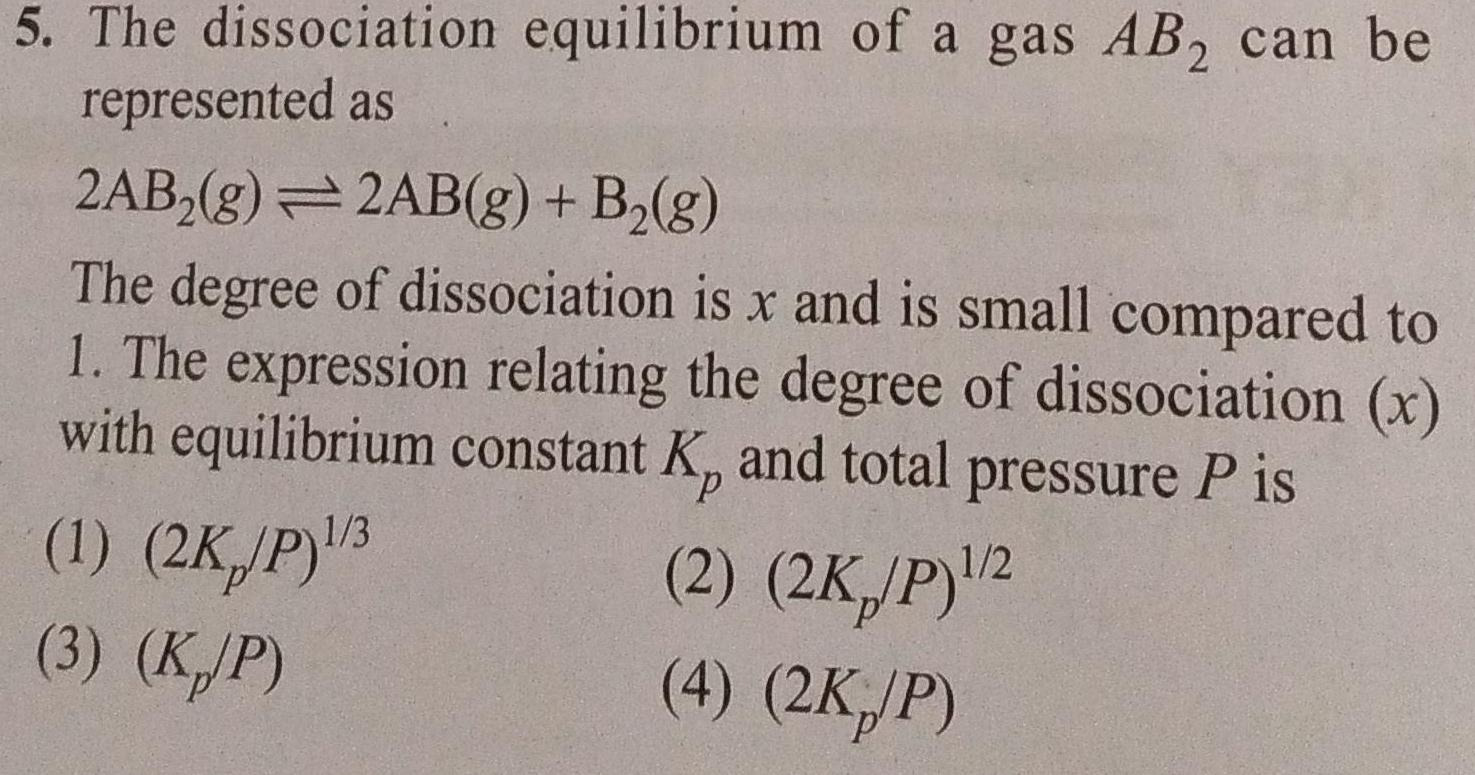Physical Chemistry
Solutions
5 The dissociation equilibrium of a gas AB can be represented as 2AB g 2AB g B g The degree of dissociation is x and is small compared to 1 The expression relating the degree of dissociation x with equilibrium constant K and total pressure P is 1 2K P 3 2 2K P 2 4 2K P 3 KJP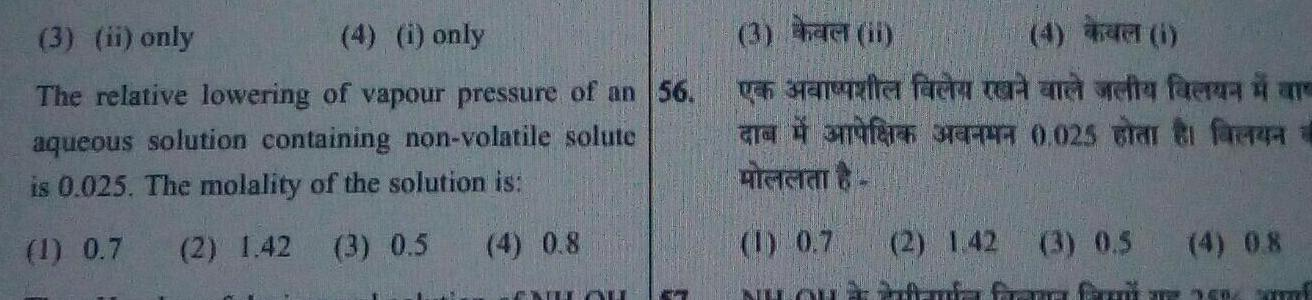Physical Chemistry
Solutions
3 ii only 4 i only The relative lowering of vapour pressure of an 56 aqueous solution containing non volatile solute is 0 025 The molality of the solution is 1 0 7 2 1 42 3 0 5 4 0 8 ENLL QU g 3 het ii 4 i Ven Sanviter facta ter and veta farza i ar fer FPT 0 025 Bar fara a FLEGE 1 0 7 2 1 42 Nunta fr 3 0 5 4 0 8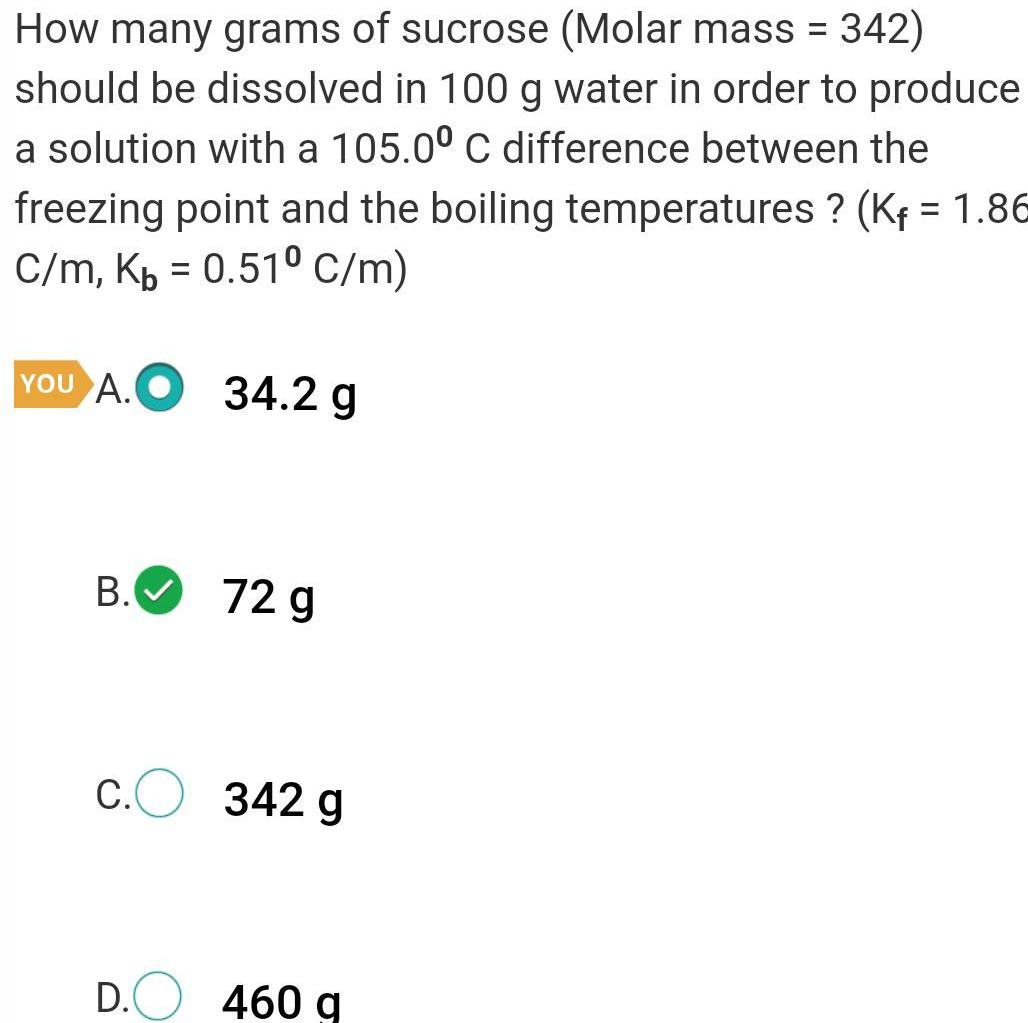Physical Chemistry
Solutions
How many grams of sucrose Molar mass 342 should be dissolved in 100 g water in order to produce a solution with a 105 0 C difference between the freezing point and the boiling temperatures Kf 1 86 C m Kp 0 51 C m YOU A 34 2 g B 72 g c 342 g D 460 a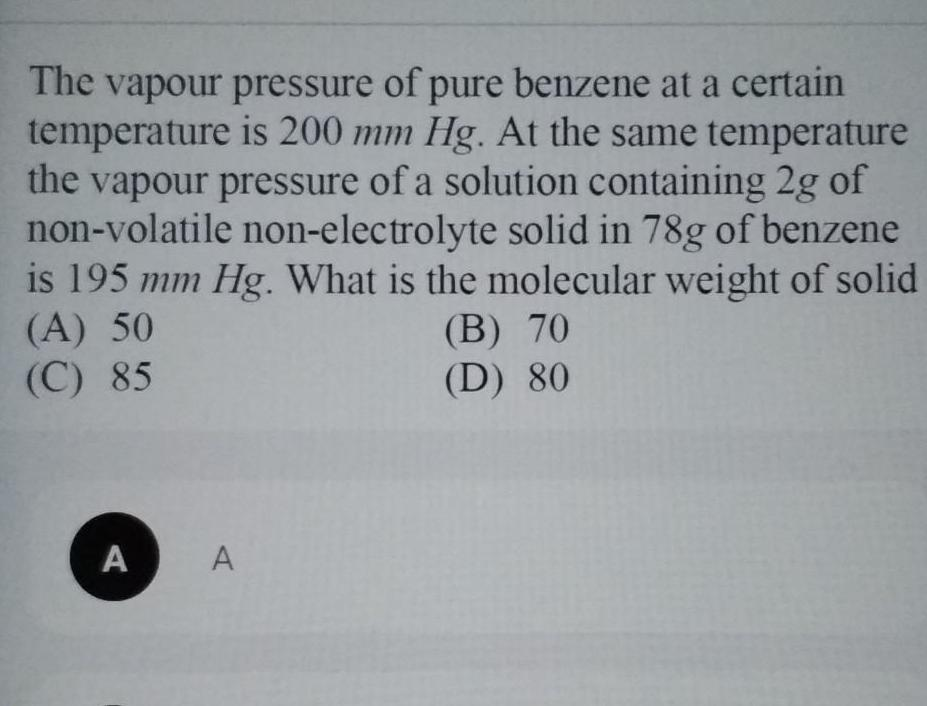Physical Chemistry
Solutions
The vapour pressure of pure benzene at a certain temperature is 200 mm Hg At the same temperature the vapour pressure of a solution containing 2g of non volatile non electrolyte solid in 78g of benzene is 195 mm Hg What is the molecular weight of solid A 50 C 85 B 70 D 80 A A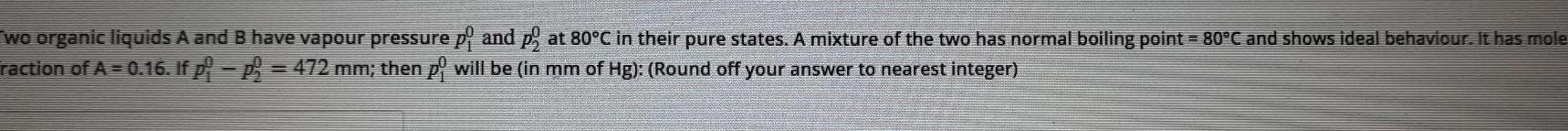Physical Chemistry
Solutions
wo organic liquids A and B have vapour pressure p and p at 80 C in their pure states A mixture of the two has normal boiling point 80 C and shows ideal behaviour It has mole raction of A 0 16 If p p 472 mm then p will be in mm of Hg Round off your answer to nearest integer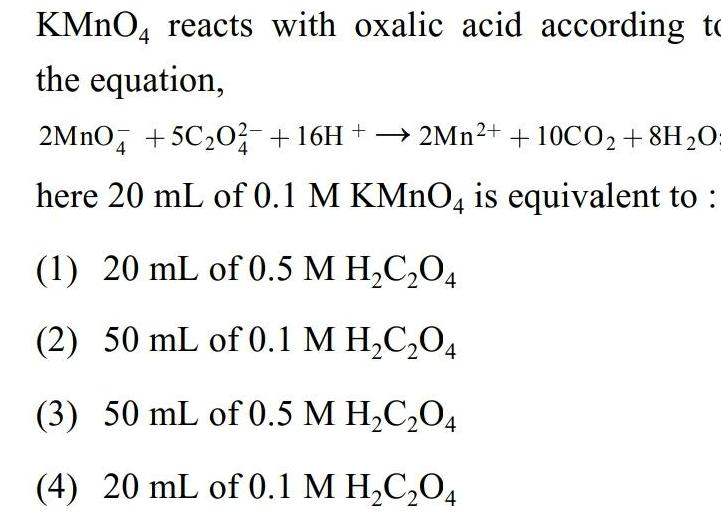Physical Chemistry
Solutions
KMnO4 reacts with oxalic acid according to the equation 2MnO4 5C 02 16H 2Mn 10CO 8H O here 20 mL of 0 1 M KMnO4 is equivalent to 1 20 mL of 0 5 M H C O4 2 50 mL of 0 1 M H C O4 3 50 mL of 0 5 M H C O4 4 20 mL of 0 1 M H C O4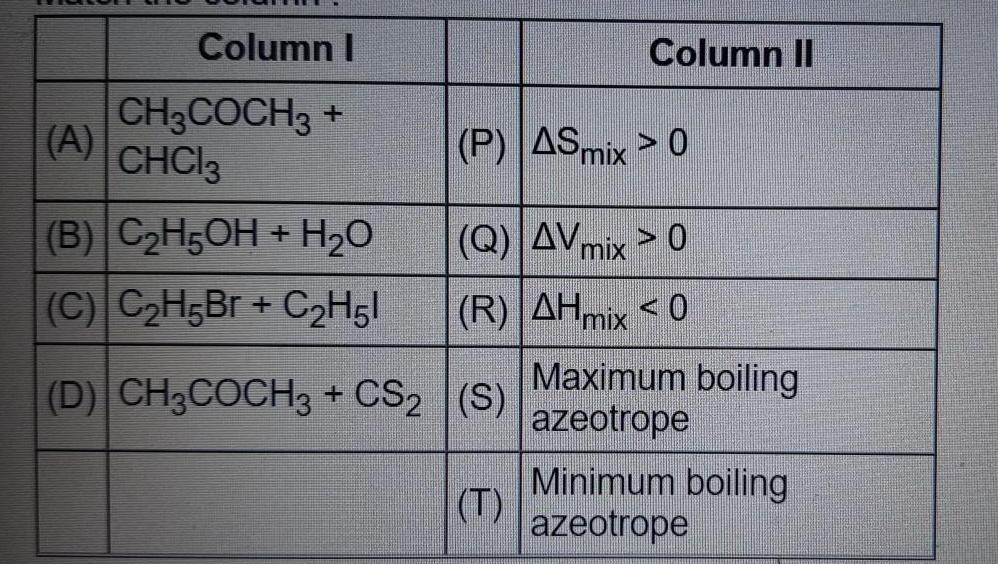Physical Chemistry
Solutions
Column I CH3COCH3 CHCl3 A B C H5OH H O C C H5Br C H51 D CH3COCH3 CS Column II P ASmix 0 Q AV mix 0 R AHmix 0 S T Maximum boiling azeotrope Minimum boiling azeotrope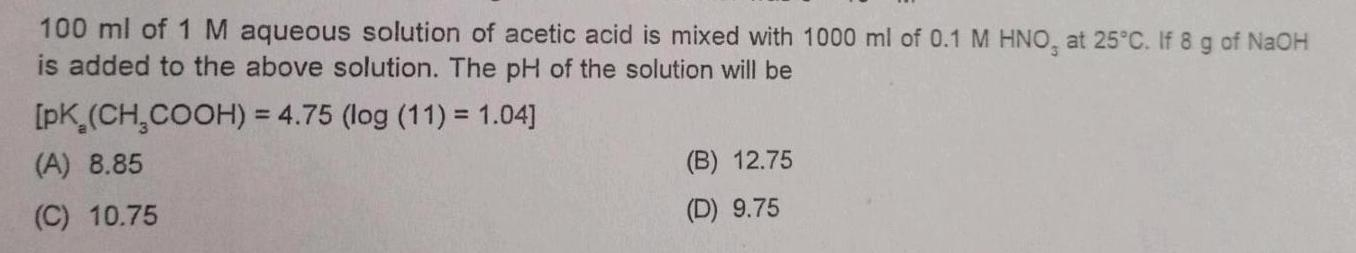Physical Chemistry
Solutions
100 ml of 1 M aqueous solution of acetic acid is mixed with 1000 ml of 0 1 M HNO at 25 C If 8 g of NaOH is added to the above solution The pH of the solution will be pK CH COOH 4 75 log 11 1 04 A 8 85 C 10 75 B 12 75 D 9 75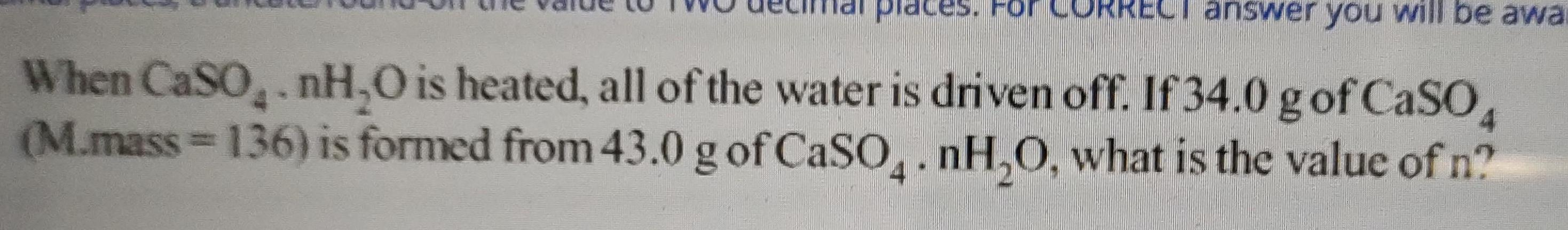Physical Chemistry
Solutions
answer you will be awa When CaSO nH O is heated all of the water is driven off If 34 0 g of CaSO4 M mass 136 is formed from 43 0 g of CaSO4 nH O what is the value of n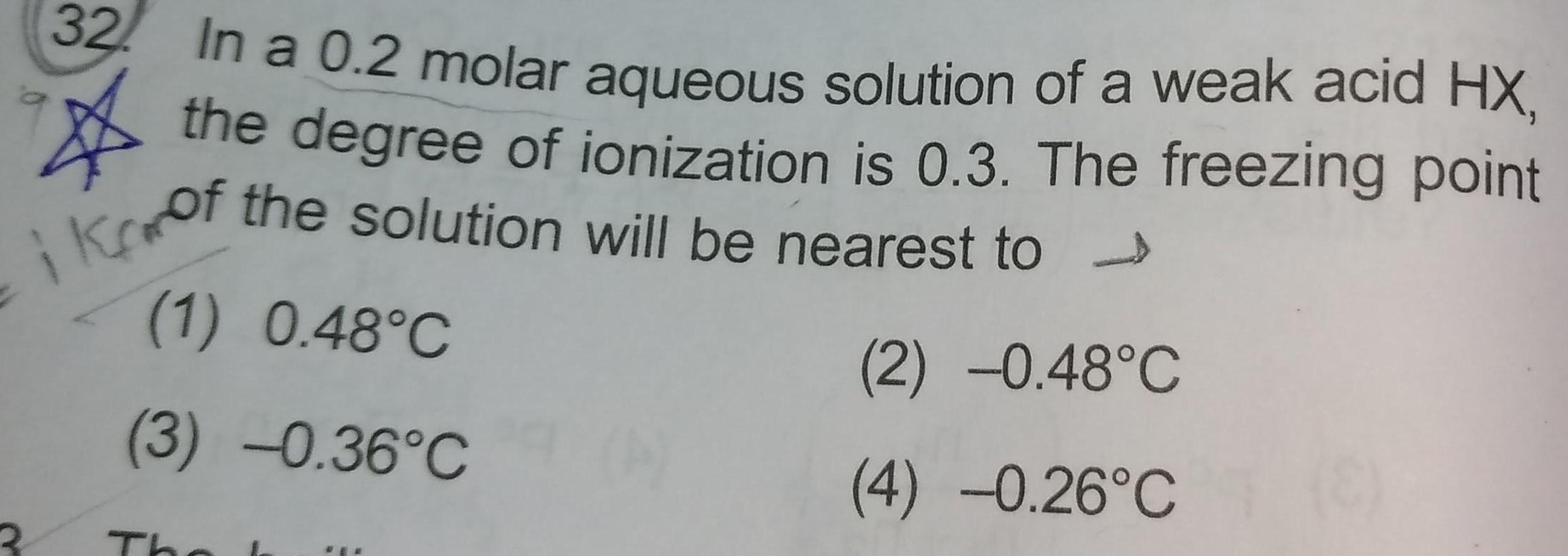Physical Chemistry
Solutions
In a 0 2 molar aqueous solution of a weak acid HX the degree of ionization is 0 3 The freezing point ikof the solution will be nearest to 1 0 48 C 3 0 36 C The I 2 0 48 C 4 0 26 C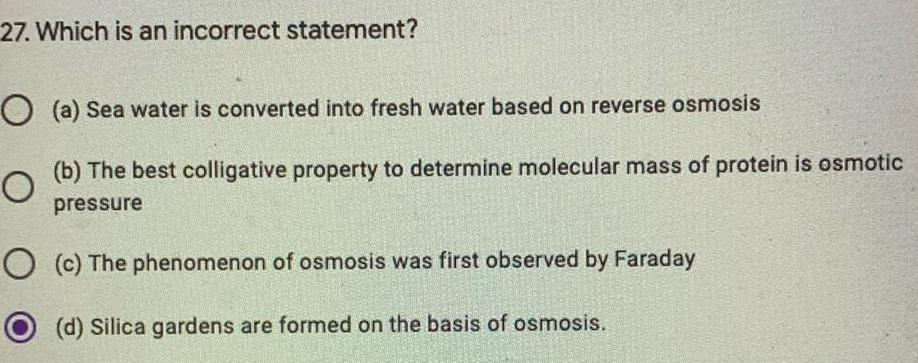Physical Chemistry
Solutions
27 Which is an incorrect statement O a Sea water is converted into fresh water based on reverse osmosis b The best colligative property to determine molecular mass of protein is osmotic O pressure O c The phenomenon of osmosis was first observed by Faraday d Silica gardens are formed on the basis of osmosis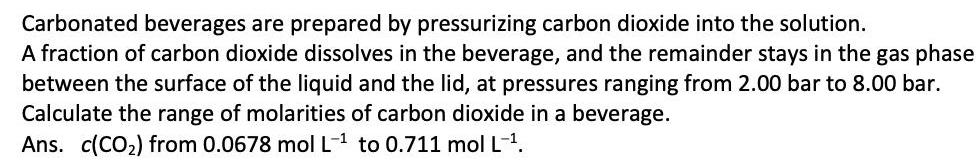Physical Chemistry
Solutions
Carbonated beverages are prepared by pressurizing carbon dioxide into the solution A fraction of carbon dioxide dissolves in the beverage and the remainder stays in the gas phase between the surface of the liquid and the lid at pressures ranging from 2 00 bar to 8 00 bar Calculate the range of molarities of carbon dioxide in a beverage Ans c CO from 0 0678 mol L to 0 711 mol L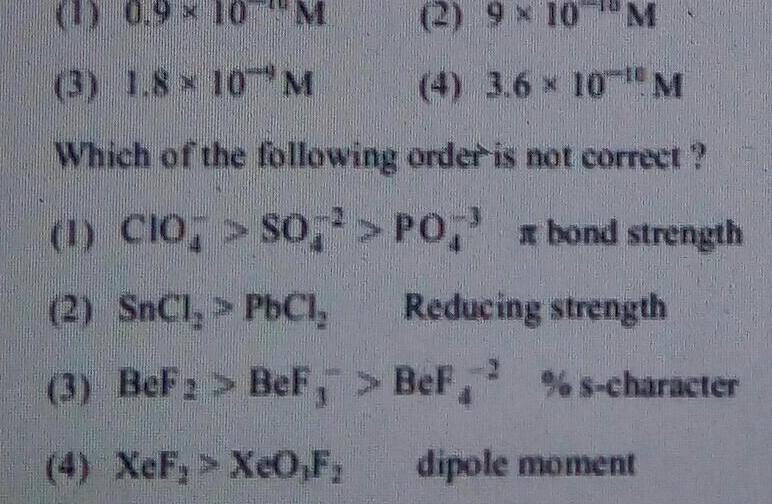Physical Chemistry
Solutions
2 9 10 M 3 1 8 10 M 4 3 6 10 M Which of the following order is not correct 1 CIO SO PO bond strength Reducing strength 2 SnCl PbCl 3 BeF BeF BeF s character 4 XeF XeO F dipole moment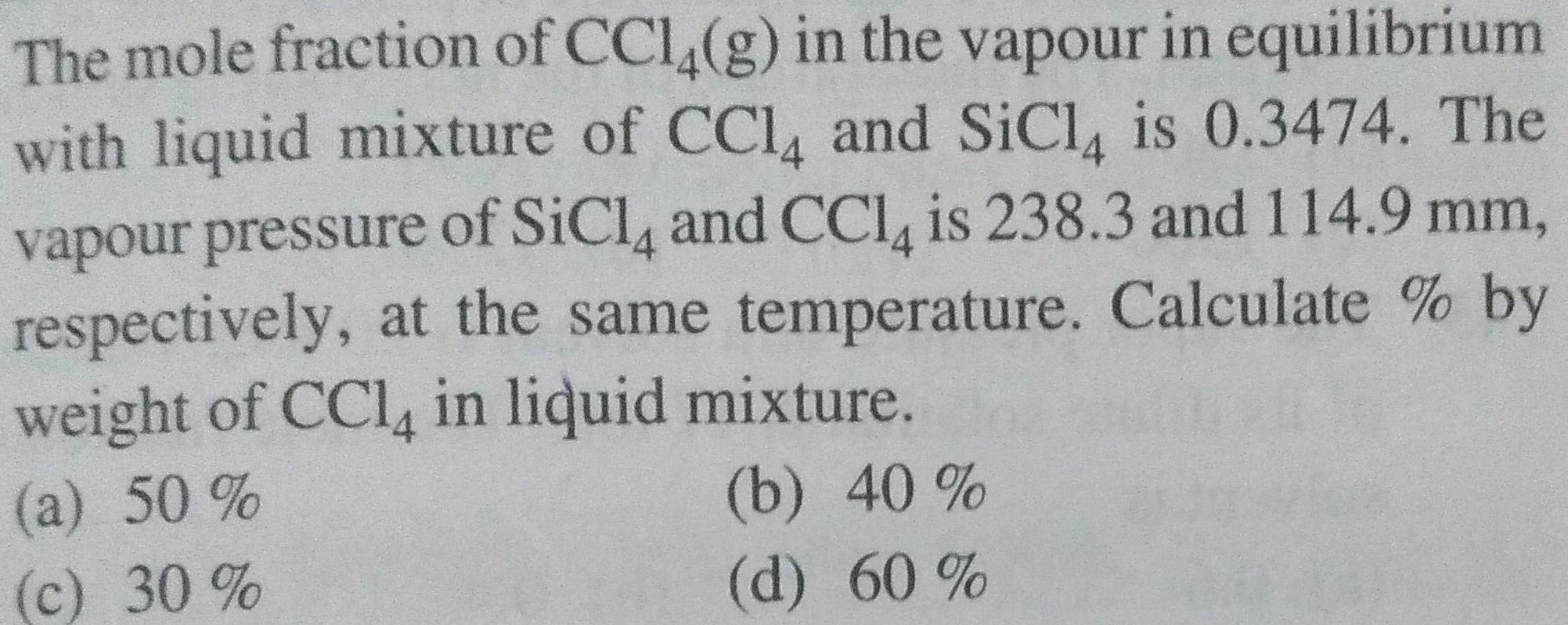Physical Chemistry
Solutions
The mole fraction of CCl4 g in the vapour in equilibrium with liquid mixture of CCl4 and SiCl4 is 0 3474 The vapour pressure of SiCl4 and CCl4 is 238 3 and 114 9 mm respectively at the same temperature Calculate by weight of CCl4 in liquid mixture a 50 c 30 b 40 d 60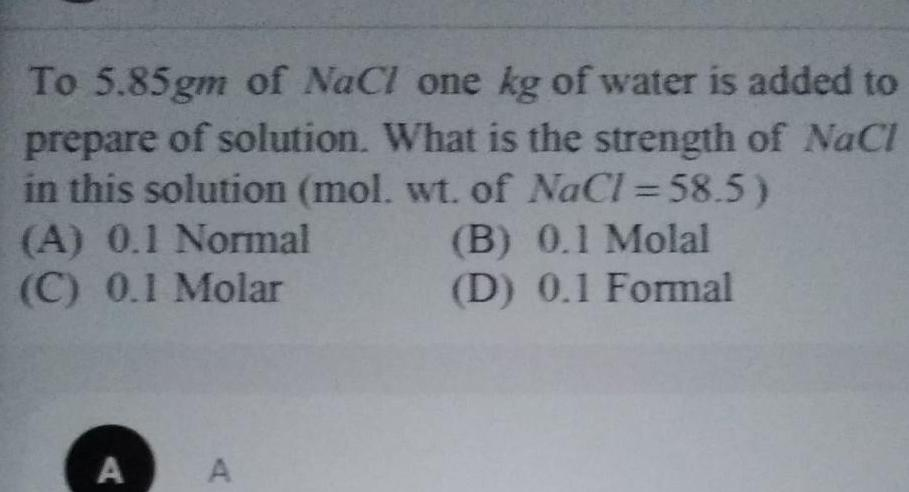Physical Chemistry
Solutions
To 5 85gm of NaCl one kg of water is added to prepare of solution What is the strength of NaCl in this solution mol wt of NaCl 58 5 A 0 1 Normal C 0 1 Molar A A B 0 1 Molal D 0 1 Formal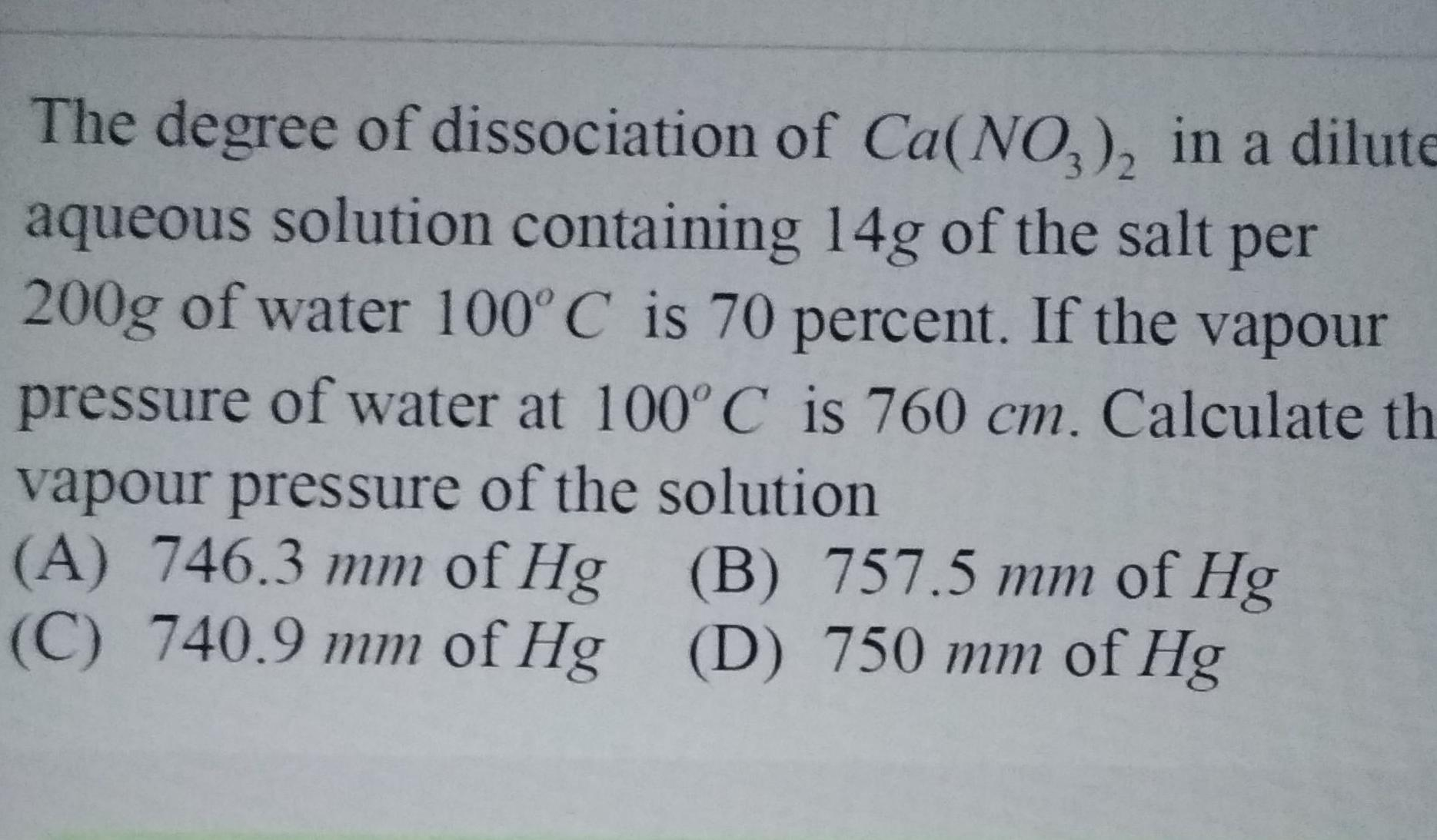Physical Chemistry
Solutions
The degree of dissociation of Ca NO3 2 in a dilute aqueous solution containing 14g of the salt per 200g of water 100 C is 70 percent If the vapour pressure of water at 100 C is 760 cm Calculate th vapour pressure of the solution A 746 3 mm of Hg C 740 9 mm of Hg B 757 5 mm of Hg D 750 mm of Hg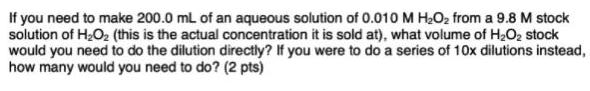Physical Chemistry
Solutions
If you need to make 200 0 mL of an aqueous solution of 0 010 M H O from a 9 8 M stock solution of H O this is the actual concentration it is sold at what volume of H O stock would you need to do the dilution directly If you were to do a series of 10x dilutions instead how many would you need to do 2 pts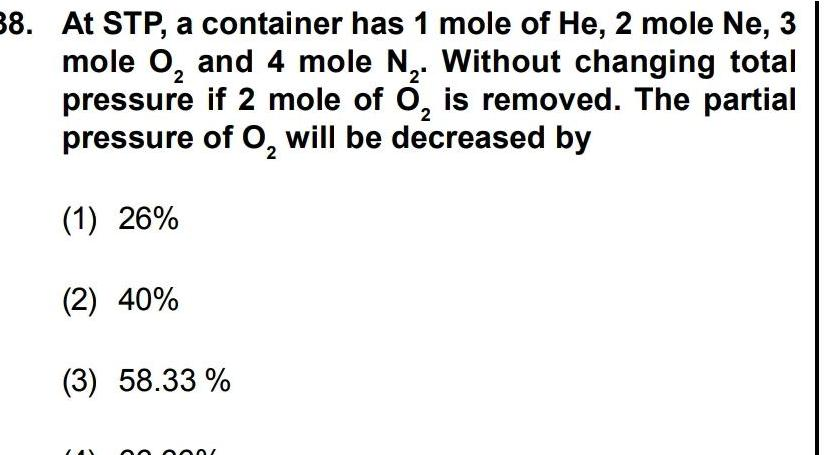Physical Chemistry
Solutions
38 At STP a container has 1 mole of He 2 mole Ne 3 2 2 mole O and 4 mole N Without changing total pressure if 2 mole of O is removed The partial of O will be decreased by 2 pressure 1 26 2 40 3 58 33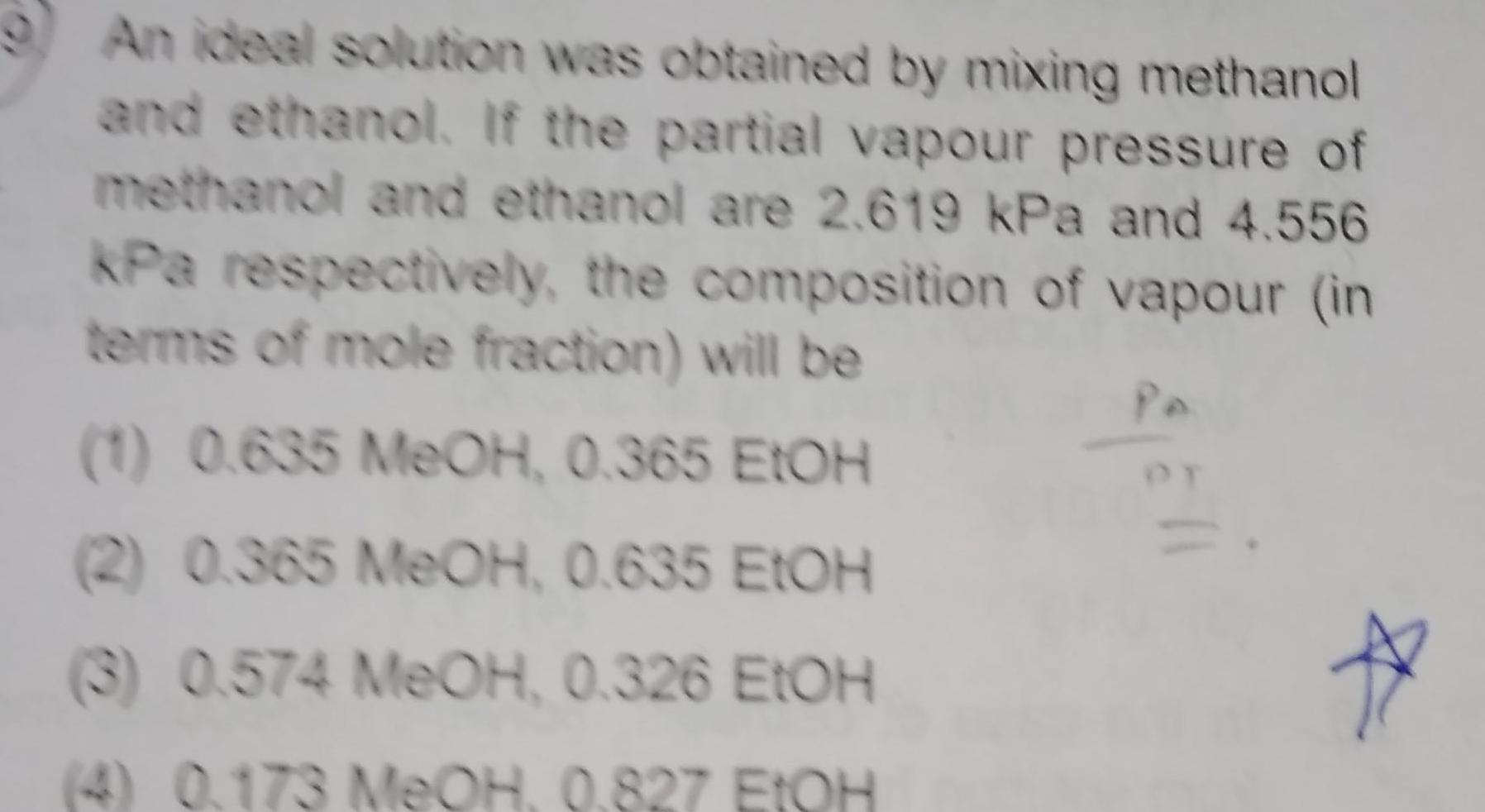Physical Chemistry
Solutions
9 An ideal solution was obtained by mixing methanol and ethanol If the partial vapour pressure of methanol and ethanol are 2 619 kPa and 4 556 kPa respectively the composition of vapour in terms of mole fraction will be 1 0 635 MeOH 0 365 EtOH 2 0 365 MeOH 0 635 EtOH 3 0 574 MeOH 0 326 EtOH 4 0 173 MeOH 0 827 EtOH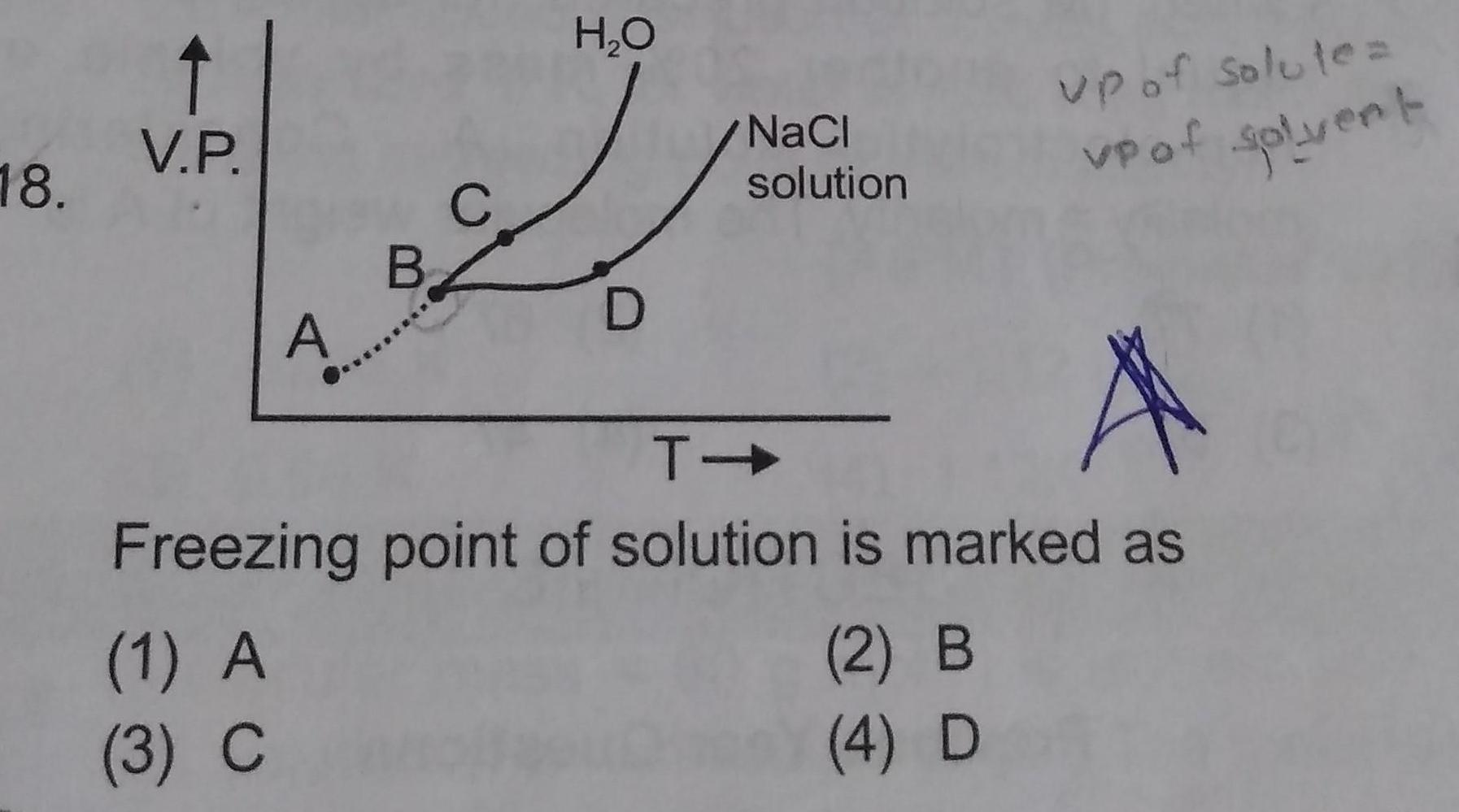Physical Chemistry
Solutions
18 V P A C B 1 A 3 C H O D NaCl solution A T Freezing point of solution is marked as up of solute vp of solvent 2 B 4 D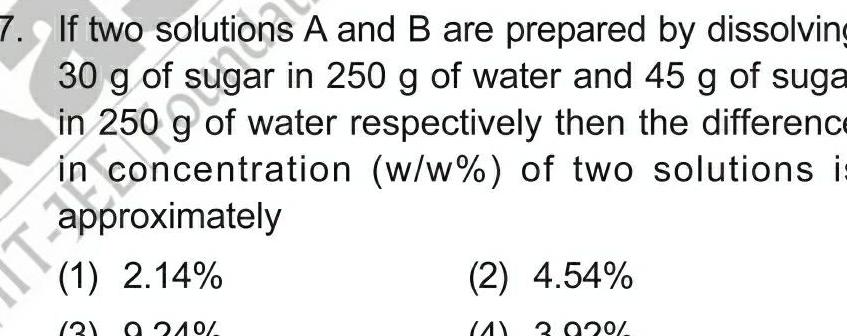Physical Chemistry
Solutions
7 If two and B are prepared by dissolving in 250 g of water and 45 g of suga in 250 g of water respectively then the difference in concentration w w of two solutions i approximately 1 2 14 3 9 21 2 4 54 3 92Physical Chemistry
Solutions
The correct relationship between molality m and molarity M of a solution is d density in g ml E m d M 1000 Ma M solute M 1000 d M solvent d M solute 1000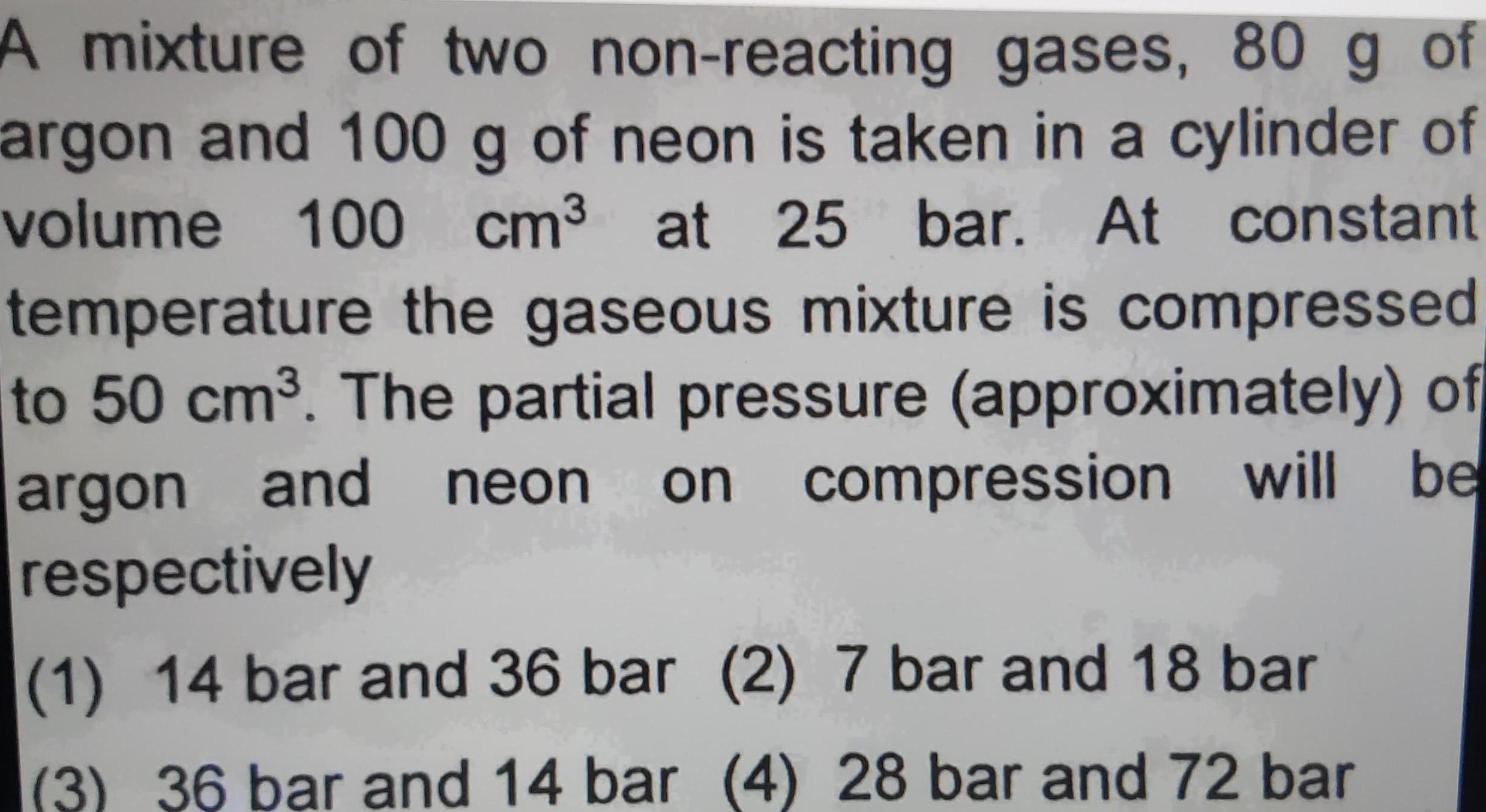Physical Chemistry
Solutions
A mixture of two non reacting gases 80 g of argon and 100 g of neon is taken in a cylinder of volume 100 cm at 25 bar At constant temperature the gaseous mixture is compressed to 50 cm The partial pressure approximately of argon and neon on compression will be respectively 1 14 bar and 36 bar 2 7 bar and 18 bar 3 36 bar and 14 bar 4 28 bar and 72 bar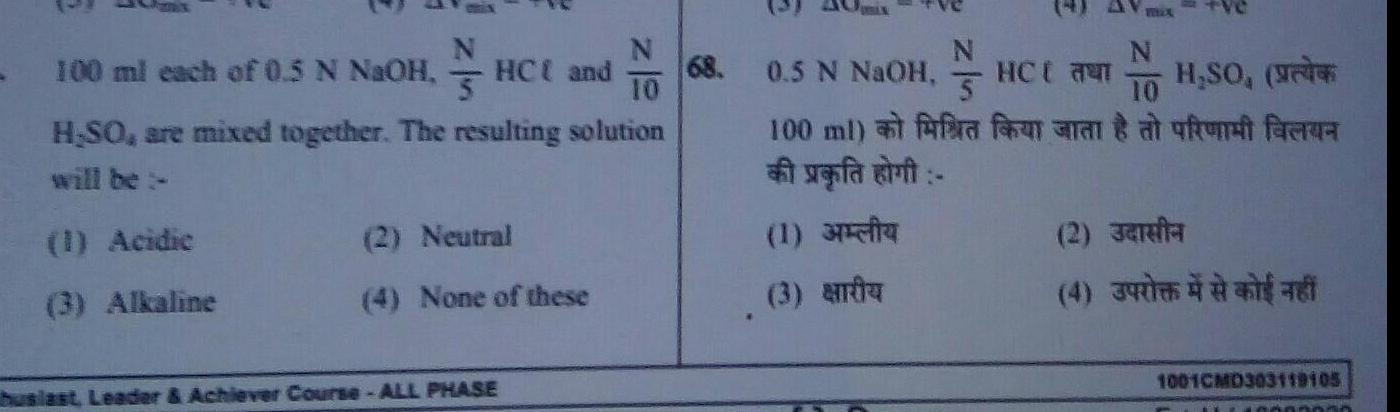Physical Chemistry
Solutions
Jeans 100 ml each of 0 5 N NaOH HC and N 5 H SO are mixed together The resulting solution will be 1 Acidic 3 Alkaline 2 Neutral 4 None of these huslast Leader Achiever Course ALL PHASE 68 N N H SO 10 100 ml fra fan arant at utrum fara vefat 0 5 N NaOH HC 5 1 3 a 2 Jarfa 4 1001CMD303119105 40003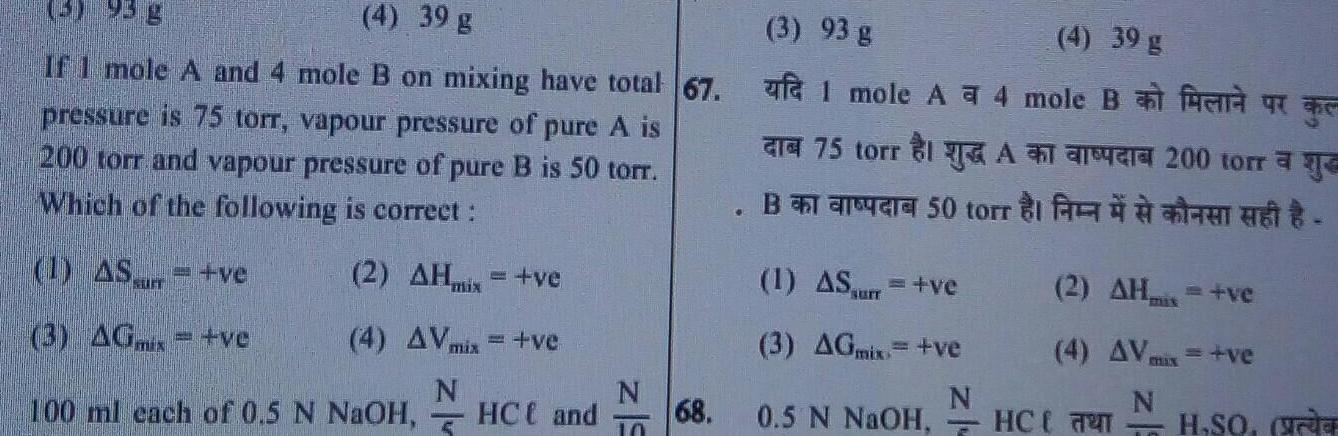Physical Chemistry
Solutions
3 95 g 4 39 g If I mole A and 4 mole B on mixing have total 67 pressure is 75 torr vapour pressure of pure A is 200 torr and vapour pressure of pure B is 50 torr Which of the following is correct 2 AHmix ve 4 AV mix ve N 5 1 AS ve 3 AGx ve 100 ml each of 0 5 N NaOH HCC and 10 68 3 93 g af 1 mole A4 mole B 75 torr B 50 torr 1 AS ve 3 AGmix ve N 0 5 N NaOH HC 4 39 g he 200 torre 2 AH ve 4 AVmax ve N T H SO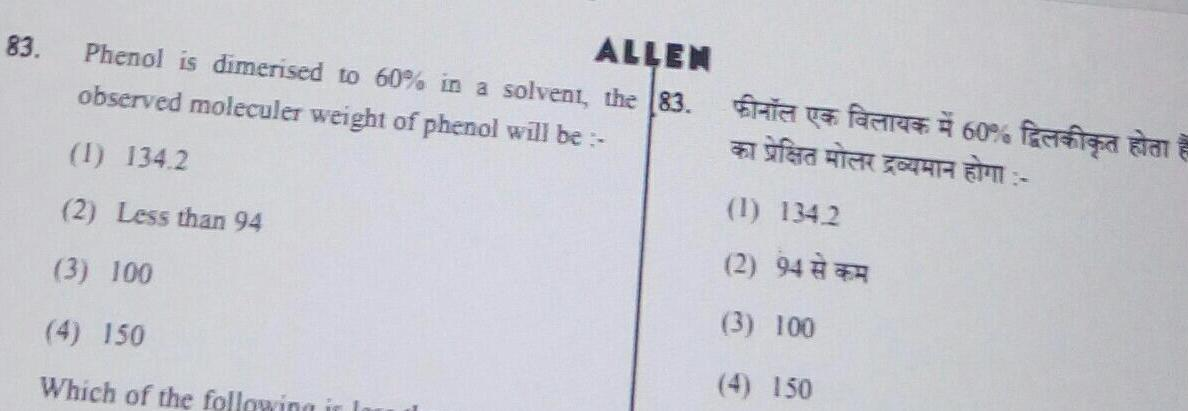Physical Chemistry
Solutions
83 ALLEN Phenol is dimerised to 60 in a solvent the 83 observed moleculer weight of phenol will be 1 134 2 2 Less than 94 3 100 4 150 Which of the following in la Sayfa WATER 1 134 2 2 94 3 100 4 150 A 60 fra a A